Скачать презентацию Review Catch-up Question Answer Outline Dear Prof

b38bf8131f0786b95440022a539463ac.ppt

• Количество слайдов: 93Review, Catch-up, Question&Answer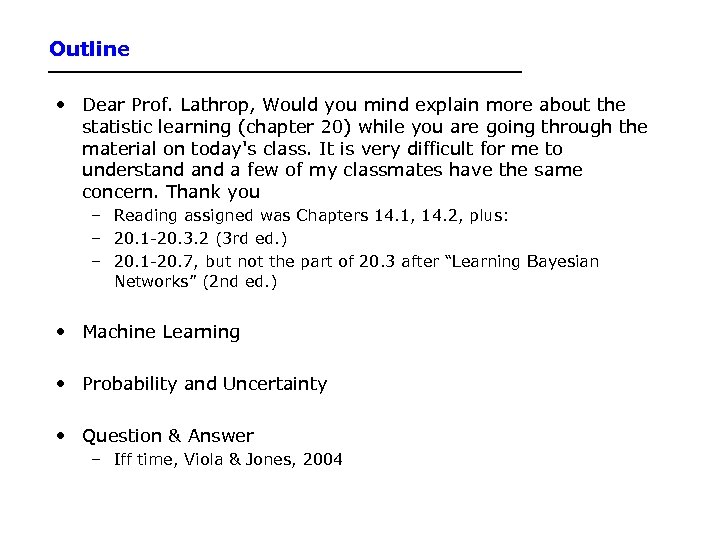Outline • Dear Prof. Lathrop, Would you mind explain more about the statistic learning (chapter 20) while you are going through the material on today's class. It is very difficult for me to understand a few of my classmates have the same concern. Thank you – Reading assigned was Chapters 14. 1, 14. 2, plus: – 20. 1 -20. 3. 2 (3 rd ed. ) – 20. 1 -20. 7, but not the part of 20. 3 after “Learning Bayesian Networks” (2 nd ed. ) • Machine Learning • Probability and Uncertainty • Question & Answer – Iff time, Viola & Jones, 2004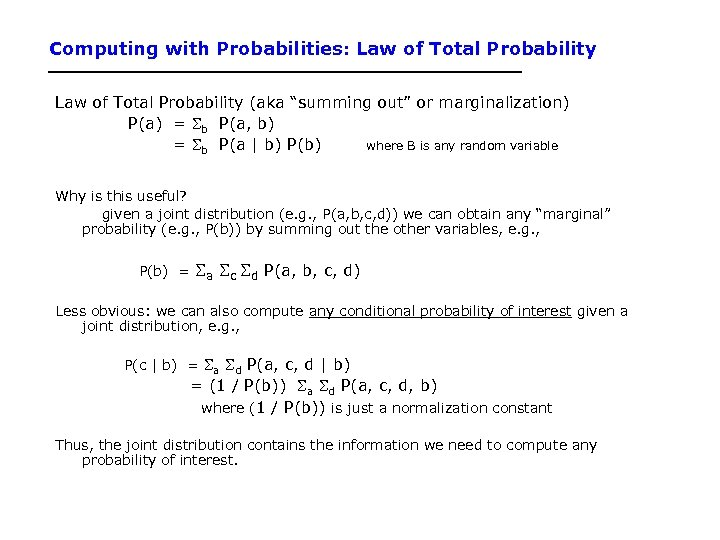Computing with Probabilities: Law of Total Probability (aka “summing out” or marginalization) P(a) = b P(a, b) = b P(a | b) P(b) where B is any random variable Why is this useful? given a joint distribution (e. g. , P(a, b, c, d)) we can obtain any “marginal” probability (e. g. , P(b)) by summing out the other variables, e. g. , P(b) = a c d P(a, b, c, d) Less obvious: we can also compute any conditional probability of interest given a joint distribution, e. g. , P(c | b) = a d P(a, c, d | b) = (1 / P(b)) a d P(a, c, d, b) where (1 / P(b)) is just a normalization constant Thus, the joint distribution contains the information we need to compute any probability of interest.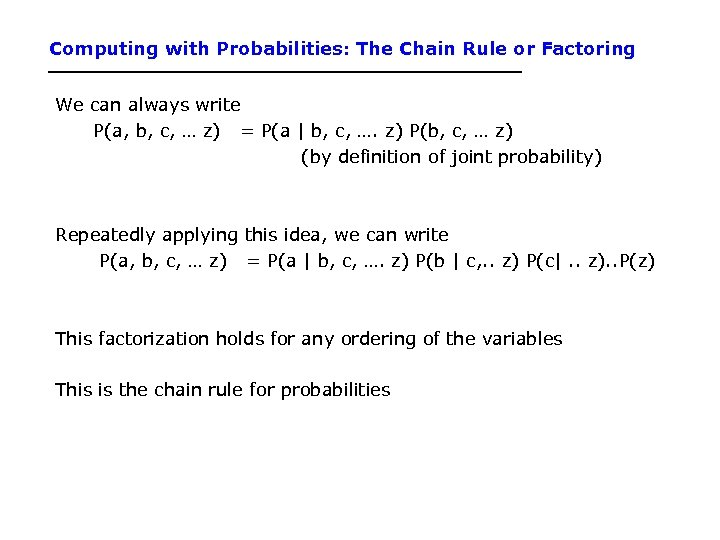Computing with Probabilities: The Chain Rule or Factoring We can always write P(a, b, c, … z) = P(a | b, c, …. z) P(b, c, … z) (by definition of joint probability) Repeatedly applying this idea, we can write P(a, b, c, … z) = P(a | b, c, …. z) P(b | c, . . z) P(c|. . z). . P(z) This factorization holds for any ordering of the variables This is the chain rule for probabilities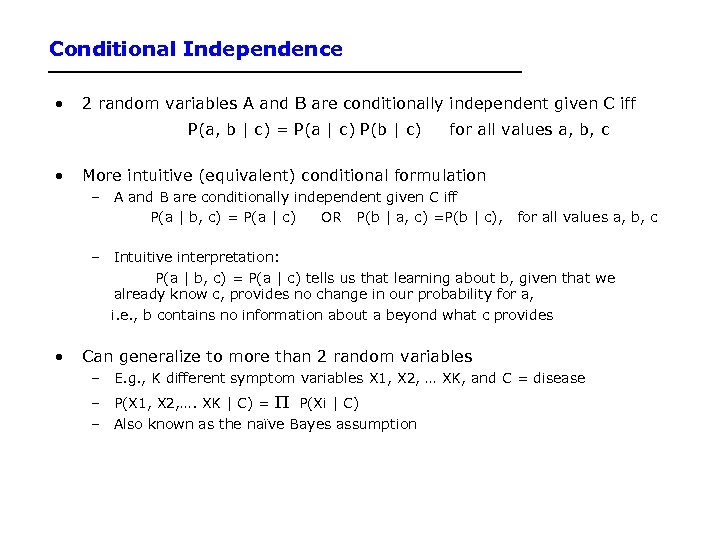Conditional Independence • 2 random variables A and B are conditionally independent given C iff P(a, b | c) = P(a | c) P(b | c) • for all values a, b, c More intuitive (equivalent) conditional formulation – A and B are conditionally independent given C iff P(a | b, c) = P(a | c) OR P(b | a, c) =P(b | c), for all values a, b, c – Intuitive interpretation: P(a | b, c) = P(a | c) tells us that learning about b, given that we already know c, provides no change in our probability for a, i. e. , b contains no information about a beyond what c provides • Can generalize to more than 2 random variables – E. g. , K different symptom variables X 1, X 2, … XK, and C = disease – P(X 1, X 2, …. XK | C) = P(Xi | C) – Also known as the naïve Bayes assumption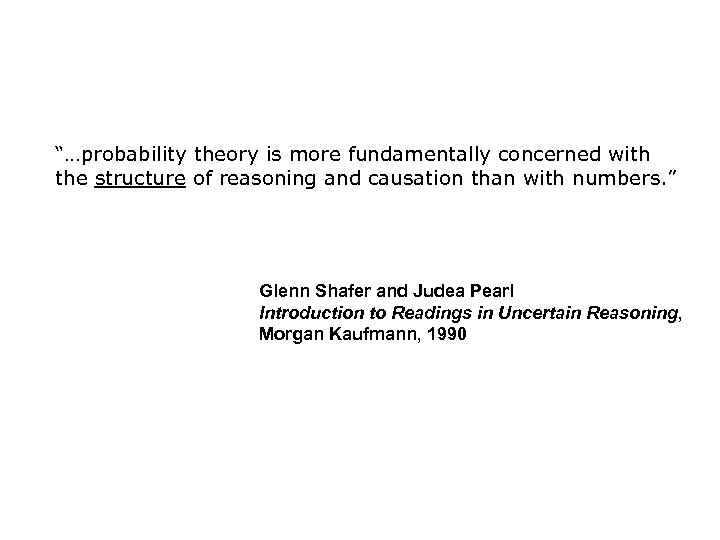“…probability theory is more fundamentally concerned with the structure of reasoning and causation than with numbers. ” Glenn Shafer and Judea Pearl Introduction to Readings in Uncertain Reasoning, Morgan Kaufmann, 1990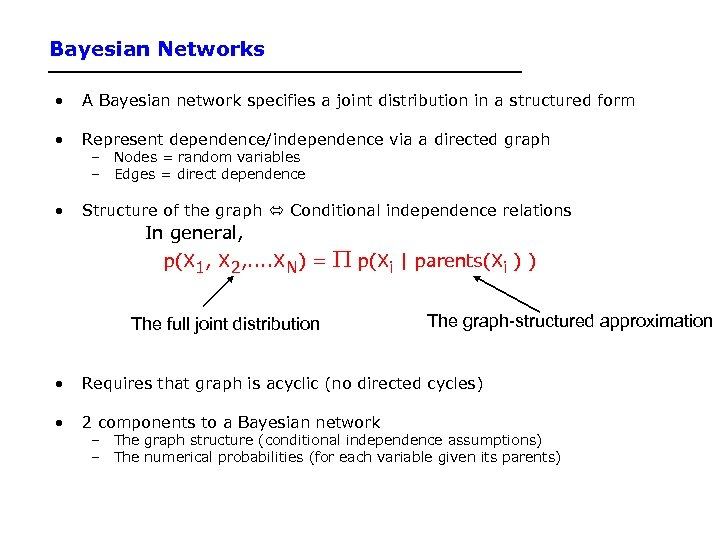Bayesian Networks • A Bayesian network specifies a joint distribution in a structured form • Represent dependence/independence via a directed graph • Structure of the graph Conditional independence relations – Nodes = random variables – Edges = direct dependence In general, p(X 1, X 2, . . XN) = p(Xi | parents(Xi ) ) The full joint distribution The graph-structured approximation • Requires that graph is acyclic (no directed cycles) • 2 components to a Bayesian network – The graph structure (conditional independence assumptions) – The numerical probabilities (for each variable given its parents)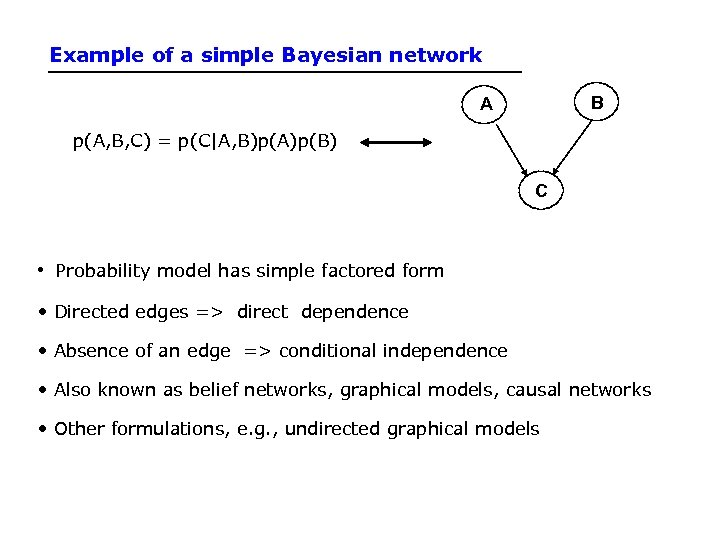Example of a simple Bayesian network B A p(A, B, C) = p(C|A, B)p(A)p(B) C • Probability model has simple factored form • Directed edges => direct dependence • Absence of an edge => conditional independence • Also known as belief networks, graphical models, causal networks • Other formulations, e. g. , undirected graphical models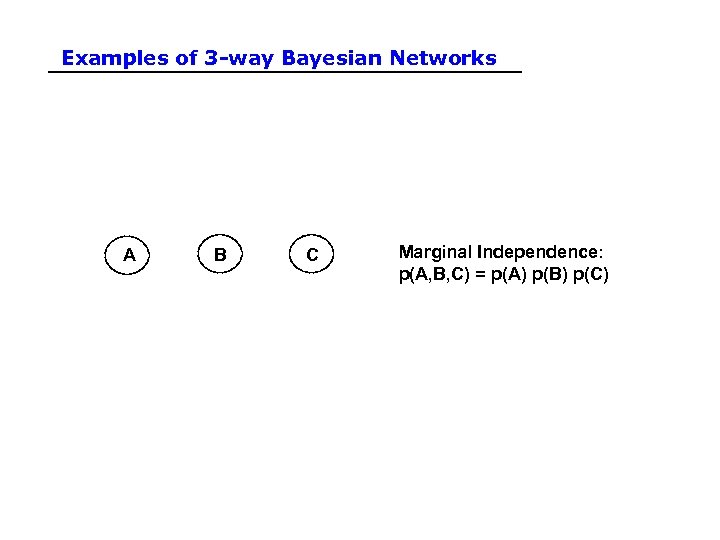Examples of 3 -way Bayesian Networks A B C Marginal Independence: p(A, B, C) = p(A) p(B) p(C)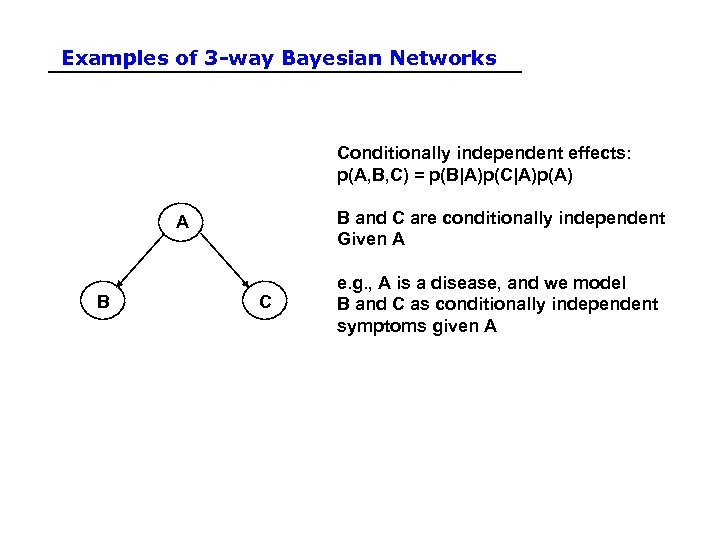Examples of 3 -way Bayesian Networks Conditionally independent effects: p(A, B, C) = p(B|A)p(C|A)p(A) B and C are conditionally independent Given A A B C e. g. , A is a disease, and we model B and C as conditionally independent symptoms given A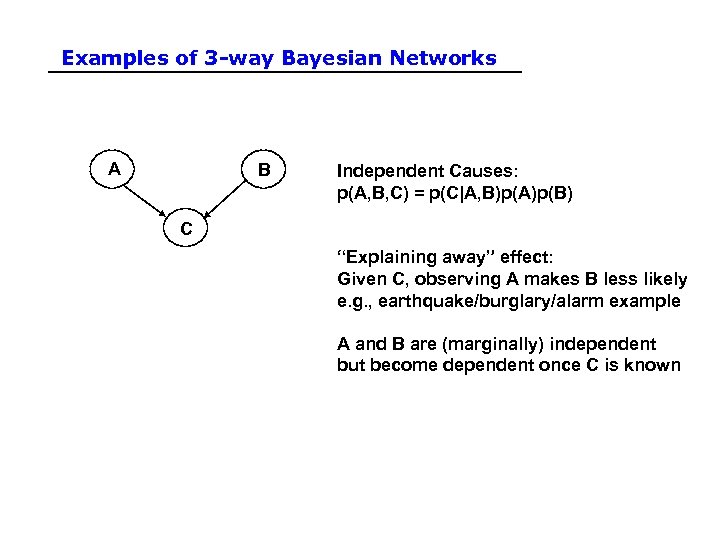Examples of 3 -way Bayesian Networks A B Independent Causes: p(A, B, C) = p(C|A, B)p(A)p(B) C “Explaining away” effect: Given C, observing A makes B less likely e. g. , earthquake/burglary/alarm example A and B are (marginally) independent but become dependent once C is known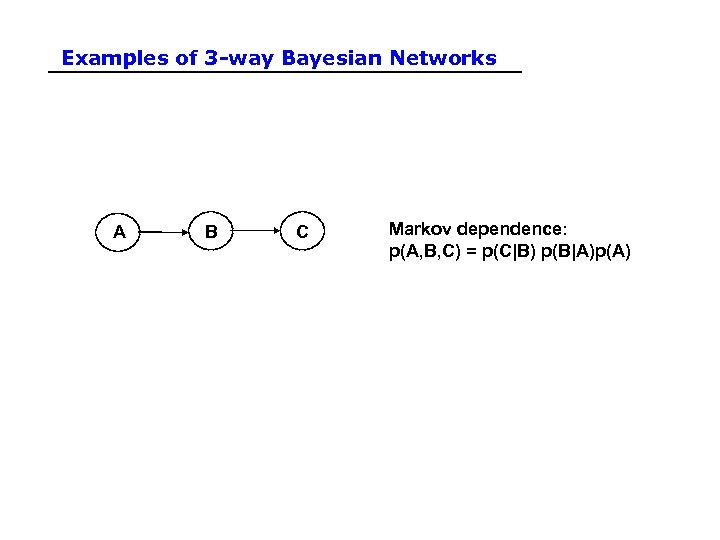Examples of 3 -way Bayesian Networks A B C Markov dependence: p(A, B, C) = p(C|B) p(B|A)p(A)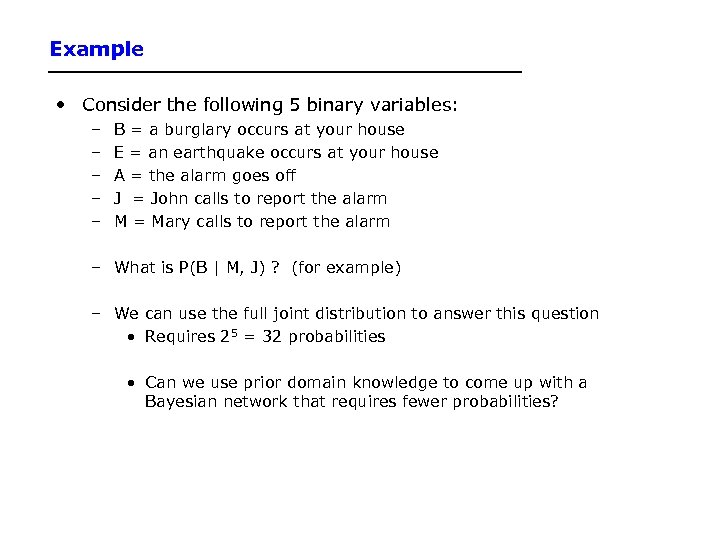Example • Consider the following 5 binary variables: – – – B = a burglary occurs at your house E = an earthquake occurs at your house A = the alarm goes off J = John calls to report the alarm M = Mary calls to report the alarm – What is P(B | M, J) ? (for example) – We can use the full joint distribution to answer this question • Requires 25 = 32 probabilities • Can we use prior domain knowledge to come up with a Bayesian network that requires fewer probabilities?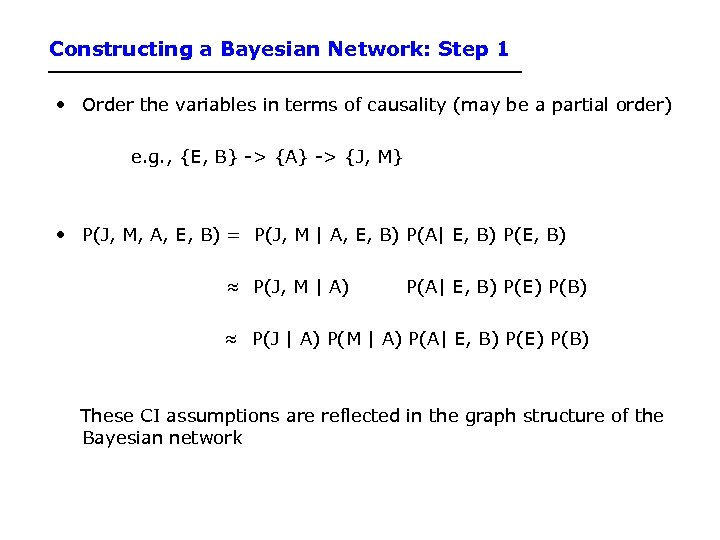Constructing a Bayesian Network: Step 1 • Order the variables in terms of causality (may be a partial order) e. g. , {E, B} -> {A} -> {J, M} • P(J, M, A, E, B) = P(J, M | A, E, B) P(A| E, B) P(E, B) ≈ P(J, M | A) P(A| E, B) P(E) P(B) ≈ P(J | A) P(M | A) P(A| E, B) P(E) P(B) These CI assumptions are reflected in the graph structure of the Bayesian network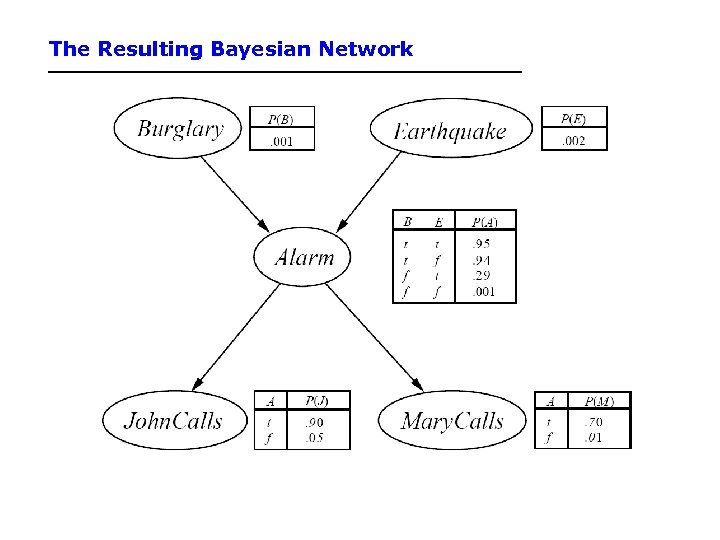The Resulting Bayesian Network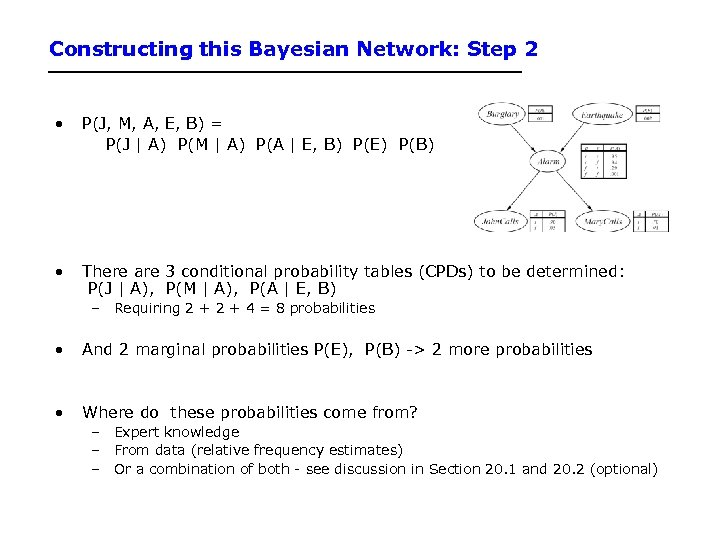Constructing this Bayesian Network: Step 2 • P(J, M, A, E, B) = P(J | A) P(M | A) P(A | E, B) P(E) P(B) • There are 3 conditional probability tables (CPDs) to be determined: P(J | A), P(M | A), P(A | E, B) – Requiring 2 + 4 = 8 probabilities • And 2 marginal probabilities P(E), P(B) -> 2 more probabilities • Where do these probabilities come from? – Expert knowledge – From data (relative frequency estimates) – Or a combination of both - see discussion in Section 20. 1 and 20. 2 (optional)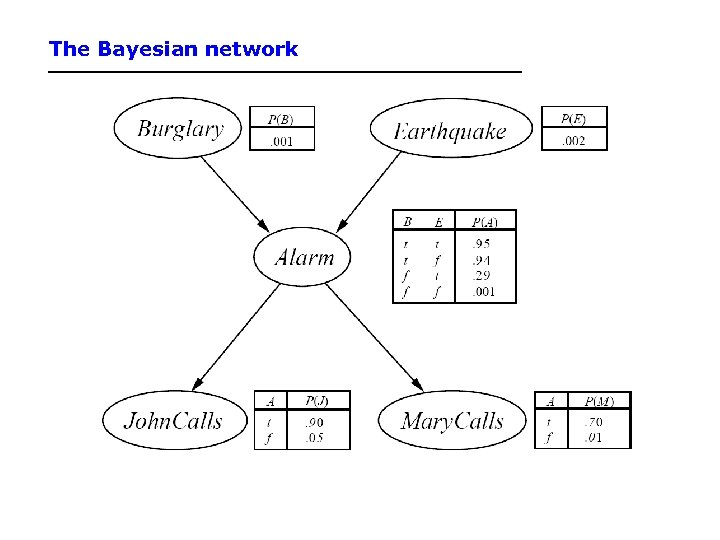The Bayesian network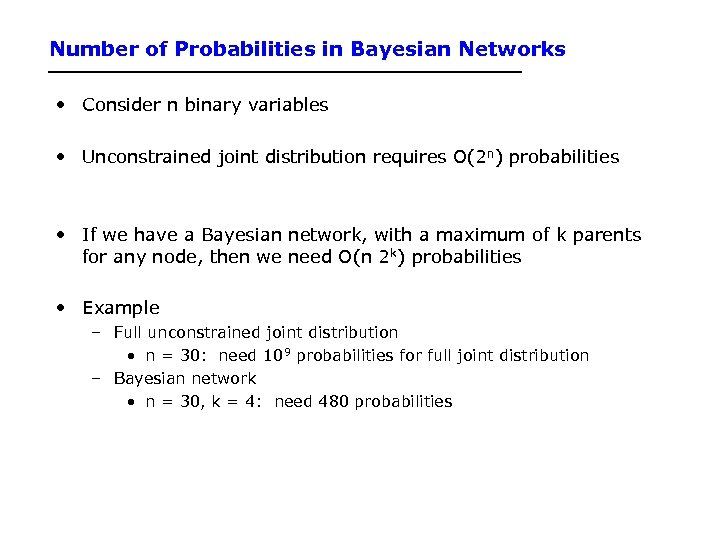Number of Probabilities in Bayesian Networks • Consider n binary variables • Unconstrained joint distribution requires O(2 n) probabilities • If we have a Bayesian network, with a maximum of k parents for any node, then we need O(n 2 k) probabilities • Example – Full unconstrained joint distribution • n = 30: need 109 probabilities for full joint distribution – Bayesian network • n = 30, k = 4: need 480 probabilities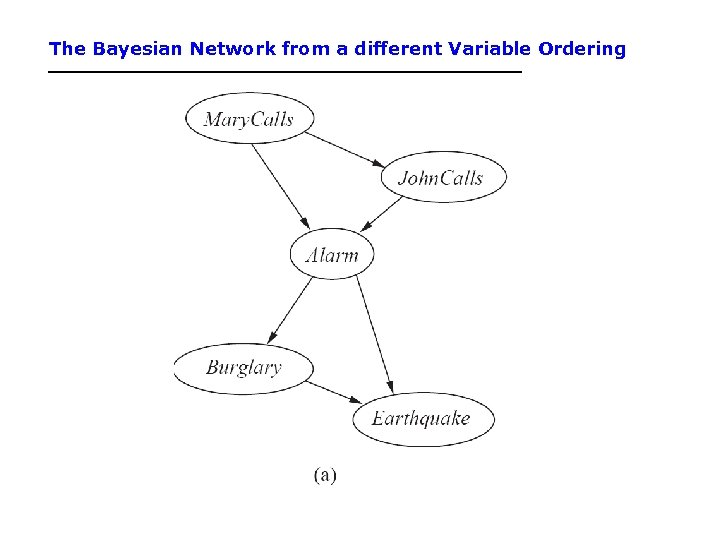The Bayesian Network from a different Variable Ordering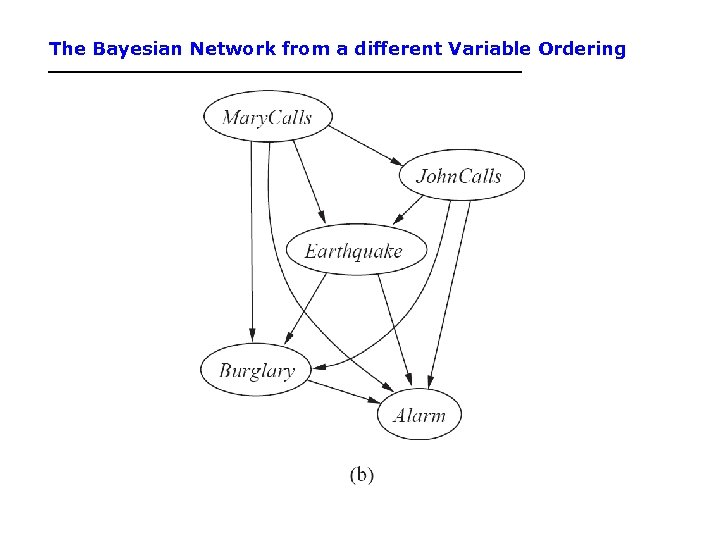The Bayesian Network from a different Variable Ordering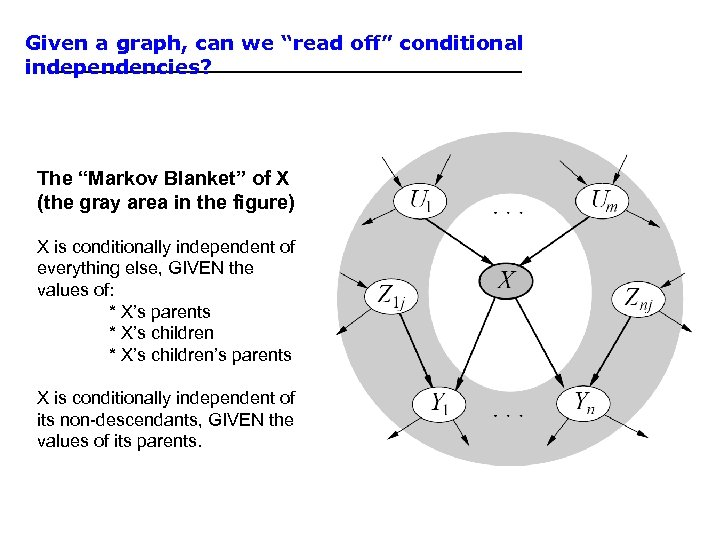Given a graph, can we “read off” conditional independencies? The “Markov Blanket” of X (the gray area in the figure) X is conditionally independent of everything else, GIVEN the values of: * X’s parents * X’s children’s parents X is conditionally independent of its non-descendants, GIVEN the values of its parents.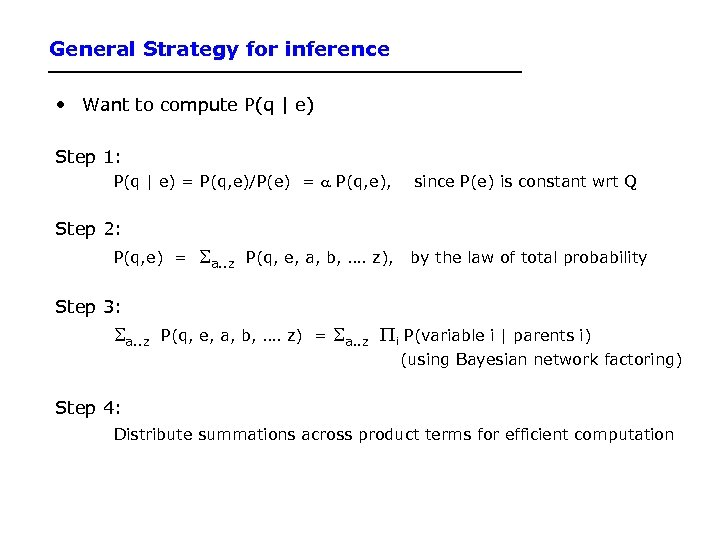General Strategy for inference • Want to compute P(q | e) Step 1: P(q | e) = P(q, e)/P(e) = a P(q, e), since P(e) is constant wrt Q Step 2: P(q, e) = a. . z P(q, e, a, b, …. z), by the law of total probability Step 3: a. . z P(q, e, a, b, …. z) = a. . z i P(variable i | parents i) (using Bayesian network factoring) Step 4: Distribute summations across product terms for efficient computation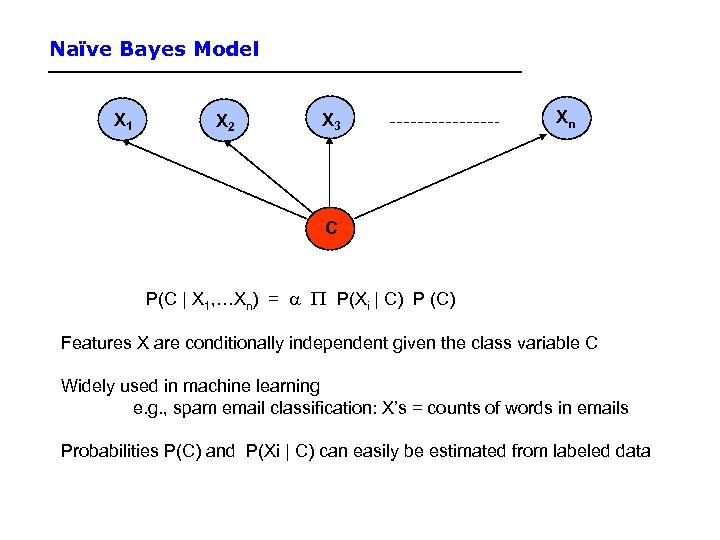Naïve Bayes Model X 1 X 2 X 3 Xn C P(C | X 1, …Xn) = a P(Xi | C) P (C) Features X are conditionally independent given the class variable C Widely used in machine learning e. g. , spam email classification: X’s = counts of words in emails Probabilities P(C) and P(Xi | C) can easily be estimated from labeled data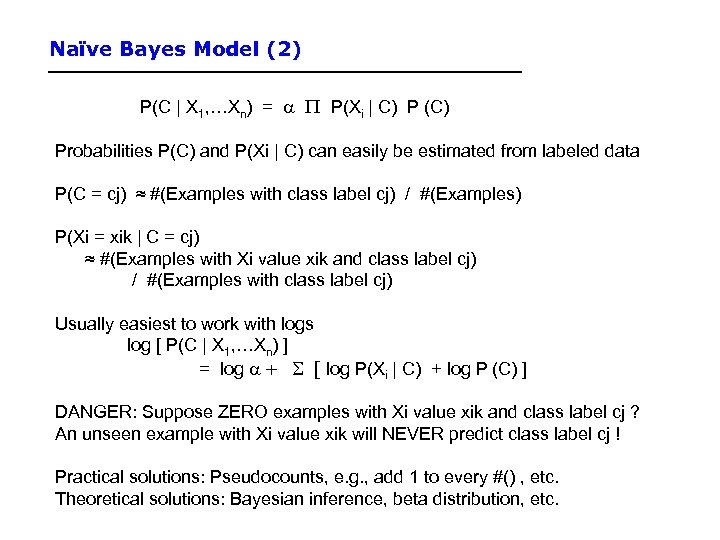Naïve Bayes Model (2) P(C | X 1, …Xn) = a P(Xi | C) P (C) Probabilities P(C) and P(Xi | C) can easily be estimated from labeled data P(C = cj) ≈ #(Examples with class label cj) / #(Examples) P(Xi = xik | C = cj) ≈ #(Examples with Xi value xik and class label cj) / #(Examples with class label cj) Usually easiest to work with logs log [ P(C | X 1, …Xn) ] = log a + [ log P(Xi | C) + log P (C) ] DANGER: Suppose ZERO examples with Xi value xik and class label cj ? An unseen example with Xi value xik will NEVER predict class label cj ! Practical solutions: Pseudocounts, e. g. , add 1 to every #() , etc. Theoretical solutions: Bayesian inference, beta distribution, etc.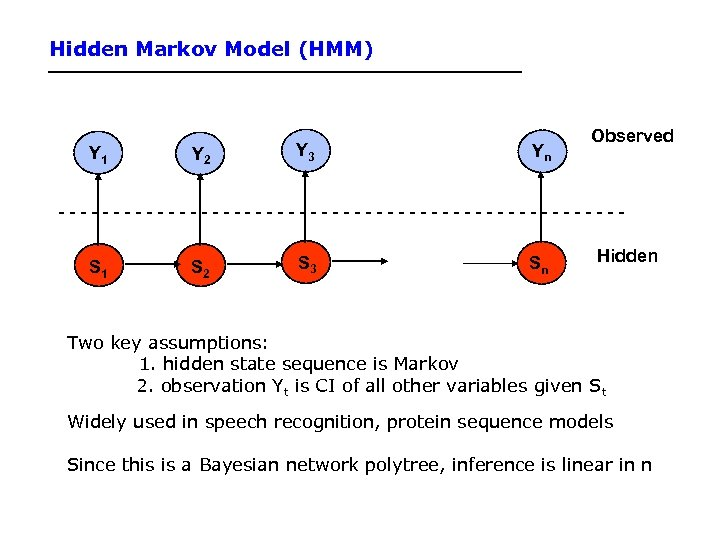Hidden Markov Model (HMM) Y 1 Y 2 Y 3 Yn Observed --------------------------S 1 S 2 S 3 Sn Hidden Two key assumptions: 1. hidden state sequence is Markov 2. observation Yt is CI of all other variables given St Widely used in speech recognition, protein sequence models Since this is a Bayesian network polytree, inference is linear in n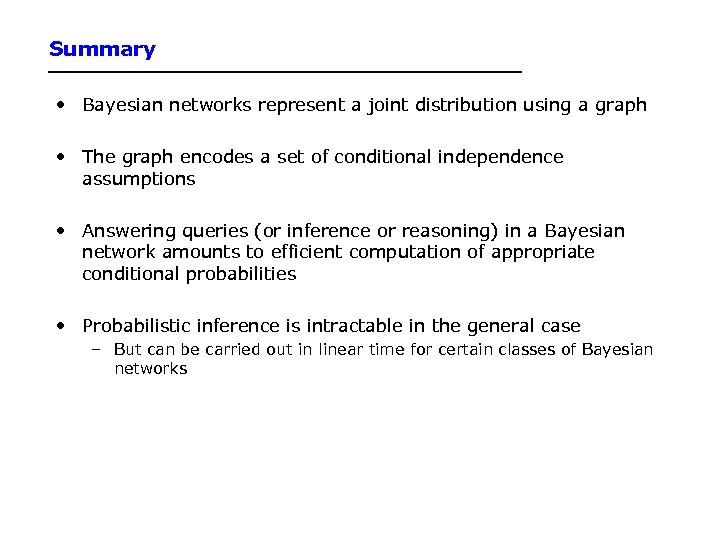Summary • Bayesian networks represent a joint distribution using a graph • The graph encodes a set of conditional independence assumptions • Answering queries (or inference or reasoning) in a Bayesian network amounts to efficient computation of appropriate conditional probabilities • Probabilistic inference is intractable in the general case – But can be carried out in linear time for certain classes of Bayesian networksOutline • Dear Prof. Lathrop, Would you mind explain more about the statistic learning (chapter 20) while you are going through the material on today's class. It is very difficult for me to understand a few of my classmates have the same concern. Thank you – Reading assigned was Chapters 14. 1, 14. 2, plus: – 20. 1 -20. 3. 2 (3 rd ed. ) – 20. 1 -20. 7, but not the part of 20. 3 after “Learning Bayesian Networks” (2 nd ed. ) • Machine Learning • Probability and Uncertainty • Question & Answer – Iff time, Viola & Jones, 2004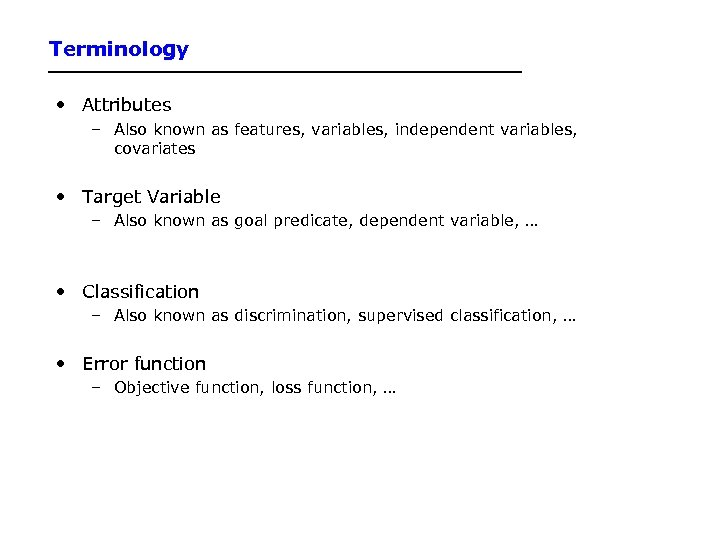Terminology • Attributes – Also known as features, variables, independent variables, covariates • Target Variable – Also known as goal predicate, dependent variable, … • Classification – Also known as discrimination, supervised classification, … • Error function – Objective function, loss function, …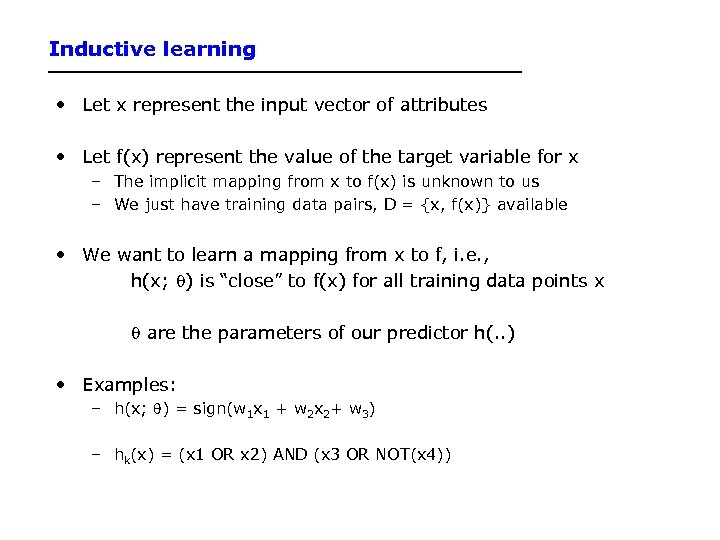Inductive learning • Let x represent the input vector of attributes • Let f(x) represent the value of the target variable for x – The implicit mapping from x to f(x) is unknown to us – We just have training data pairs, D = {x, f(x)} available • We want to learn a mapping from x to f, i. e. , h(x; q) is “close” to f(x) for all training data points x q are the parameters of our predictor h(. . ) • Examples: – h(x; q) = sign(w 1 x 1 + w 2 x 2+ w 3) – hk(x) = (x 1 OR x 2) AND (x 3 OR NOT(x 4))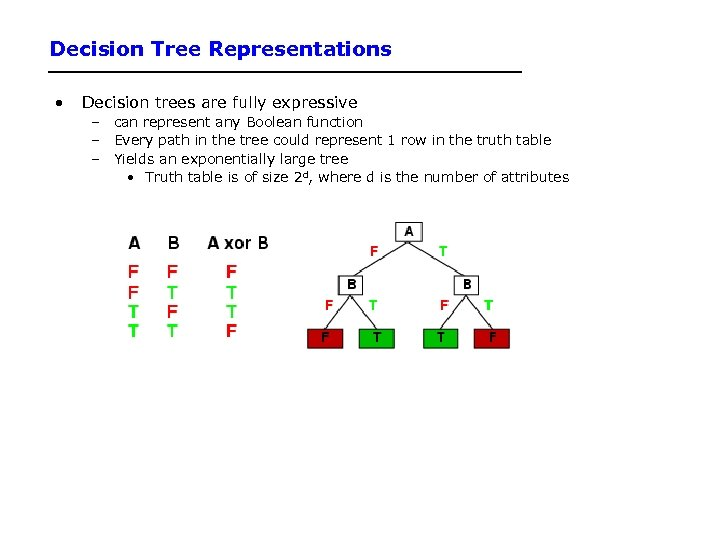Decision Tree Representations • Decision trees are fully expressive – can represent any Boolean function – Every path in the tree could represent 1 row in the truth table – Yields an exponentially large tree • Truth table is of size 2 d, where d is the number of attributes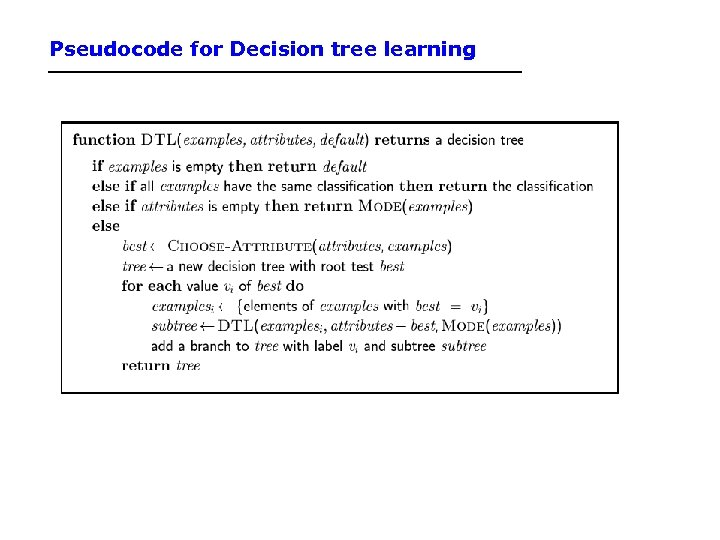Pseudocode for Decision tree learning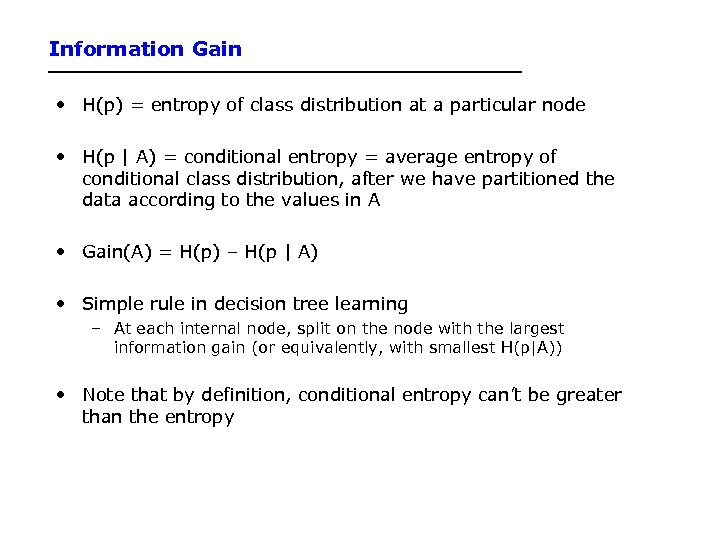Information Gain • H(p) = entropy of class distribution at a particular node • H(p | A) = conditional entropy = average entropy of conditional class distribution, after we have partitioned the data according to the values in A • Gain(A) = H(p) – H(p | A) • Simple rule in decision tree learning – At each internal node, split on the node with the largest information gain (or equivalently, with smallest H(p|A)) • Note that by definition, conditional entropy can’t be greater than the entropy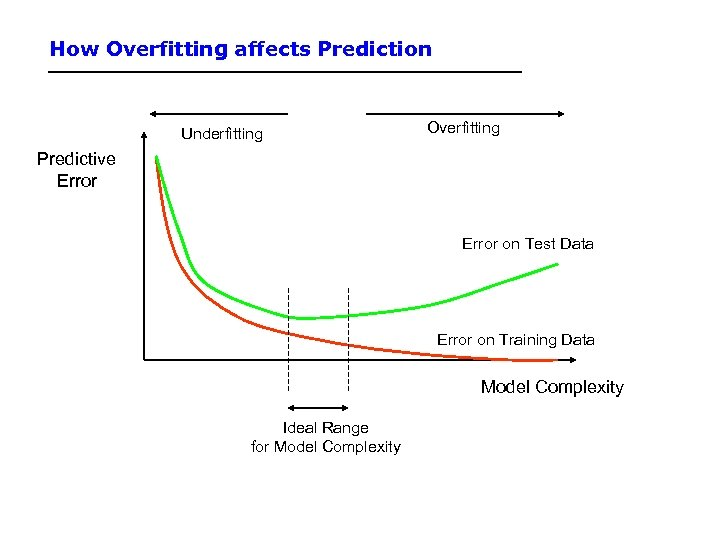How Overfitting affects Prediction Underfitting Overfitting Predictive Error on Test Data Error on Training Data Model Complexity Ideal Range for Model Complexity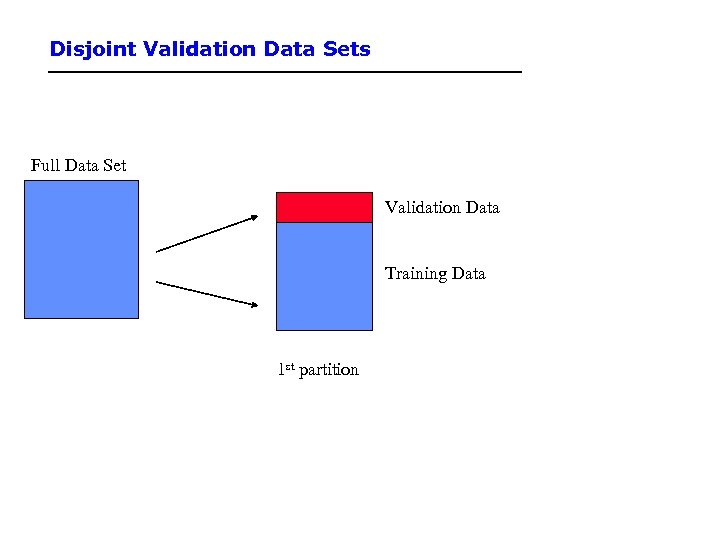Disjoint Validation Data Sets Full Data Set Validation Data Training Data 1 st partition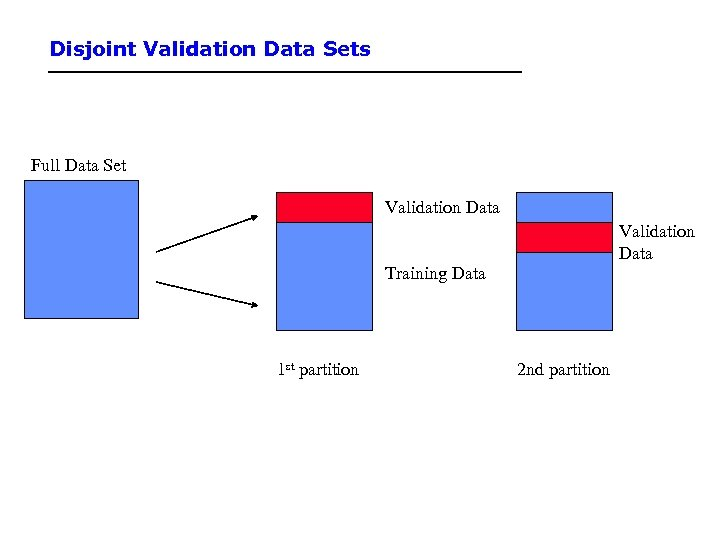Disjoint Validation Data Sets Full Data Set Validation Data Training Data 1 st partition 2 nd partition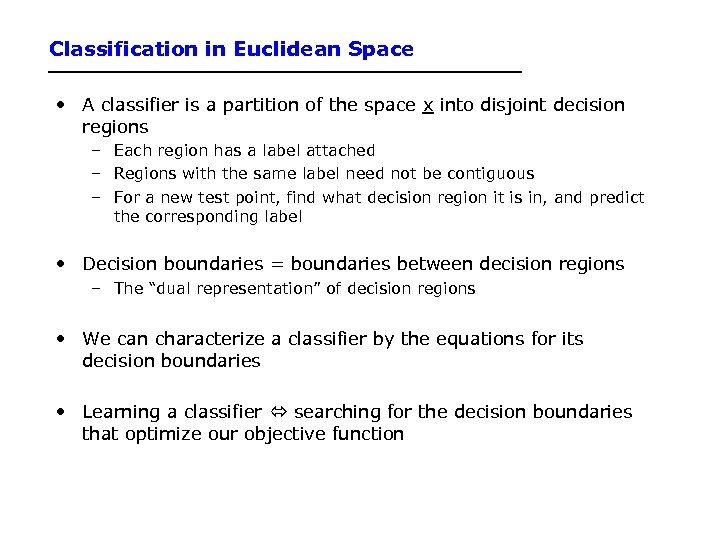Classification in Euclidean Space • A classifier is a partition of the space x into disjoint decision regions – Each region has a label attached – Regions with the same label need not be contiguous – For a new test point, find what decision region it is in, and predict the corresponding label • Decision boundaries = boundaries between decision regions – The “dual representation” of decision regions • We can characterize a classifier by the equations for its decision boundaries • Learning a classifier searching for the decision boundaries that optimize our objective function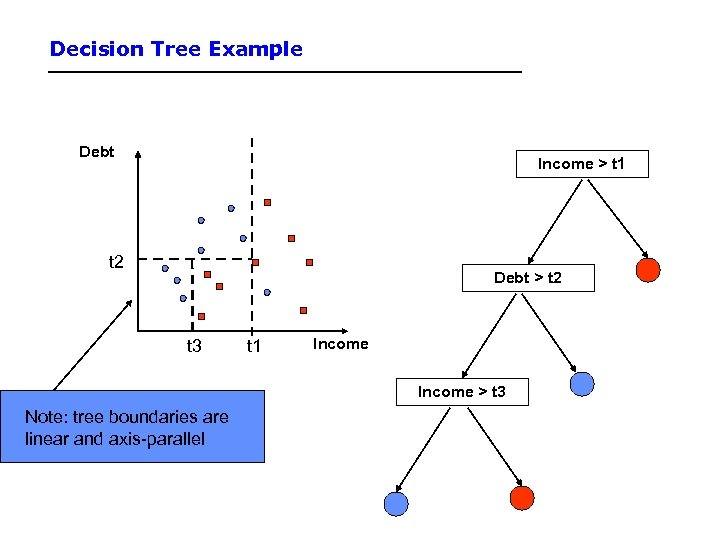Decision Tree Example Debt Income > t 1 t 2 Debt > t 2 t 3 t 1 Income > t 3 Note: tree boundaries are linear and axis-parallel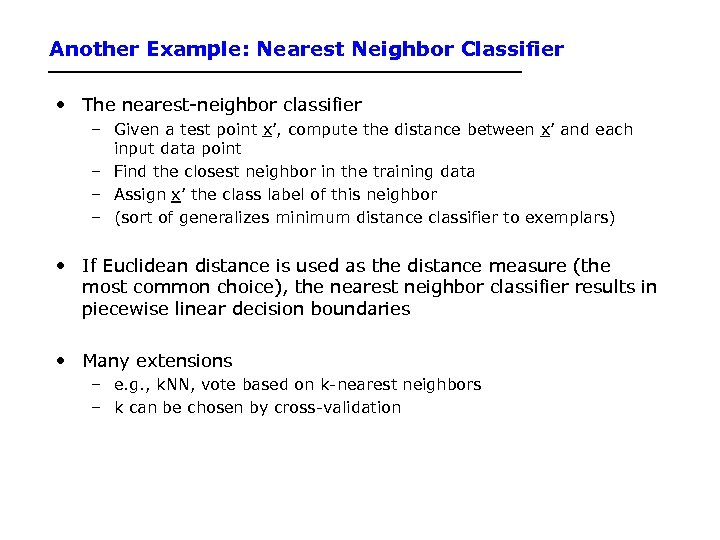Another Example: Nearest Neighbor Classifier • The nearest-neighbor classifier – Given a test point x’, compute the distance between x’ and each input data point – Find the closest neighbor in the training data – Assign x’ the class label of this neighbor – (sort of generalizes minimum distance classifier to exemplars) • If Euclidean distance is used as the distance measure (the most common choice), the nearest neighbor classifier results in piecewise linear decision boundaries • Many extensions – e. g. , k. NN, vote based on k-nearest neighbors – k can be chosen by cross-validation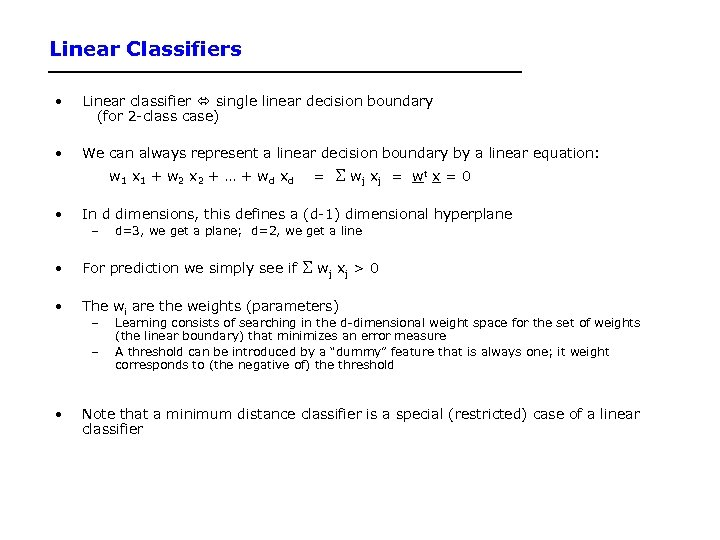Linear Classifiers • Linear classifier single linear decision boundary (for 2 -class case) • We can always represent a linear decision boundary by a linear equation: w 1 x 1 + w 2 x 2 + … + w d xd • = wj x j = wt x = 0 In d dimensions, this defines a (d-1) dimensional hyperplane – d=3, we get a plane; d=2, we get a line wj x j > 0 • For prediction we simply see if • The wi are the weights (parameters) – – • Learning consists of searching in the d-dimensional weight space for the set of weights (the linear boundary) that minimizes an error measure A threshold can be introduced by a “dummy” feature that is always one; it weight corresponds to (the negative of) the threshold Note that a minimum distance classifier is a special (restricted) case of a linear classifier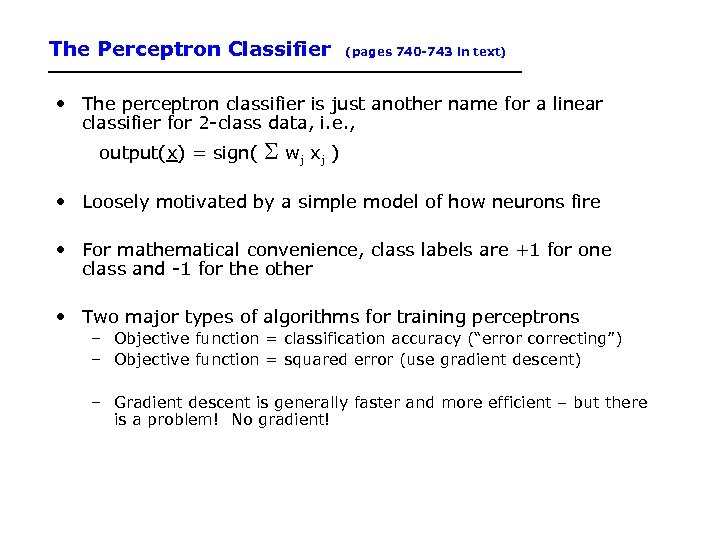The Perceptron Classifier (pages 740 -743 in text) • The perceptron classifier is just another name for a linear classifier for 2 -class data, i. e. , output(x) = sign( wj x j ) • Loosely motivated by a simple model of how neurons fire • For mathematical convenience, class labels are +1 for one class and -1 for the other • Two major types of algorithms for training perceptrons – Objective function = classification accuracy (“error correcting”) – Objective function = squared error (use gradient descent) – Gradient descent is generally faster and more efficient – but there is a problem! No gradient!Two different types of perceptron output x-axis below is f(x) = f = weighted sum of inputs y-axis is the perceptron output o(f) Thresholded output, takes values +1 or -1 f s(f) f Sigmoid output, takes real values between -1 and +1 The sigmoid is in effect an approximation to the threshold function above, but has a gradient that we can use for learning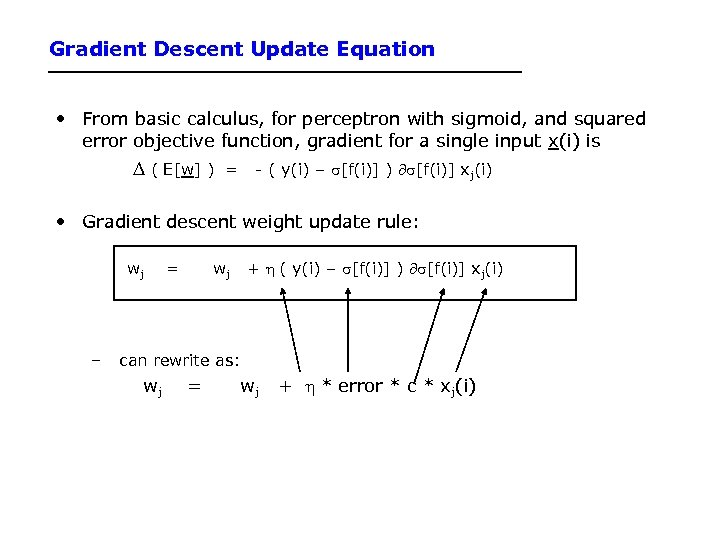Gradient Descent Update Equation • From basic calculus, for perceptron with sigmoid, and squared error objective function, gradient for a single input x(i) is D ( E[w] ) = - ( y(i) – s[f(i)] ) ¶s[f(i)] xj(i) • Gradient descent weight update rule: wj – = wj + h ( y(i) – s[f(i)] ) ¶s[f(i)] xj(i) can rewrite as: wj = wj + h * error * c * xj(i)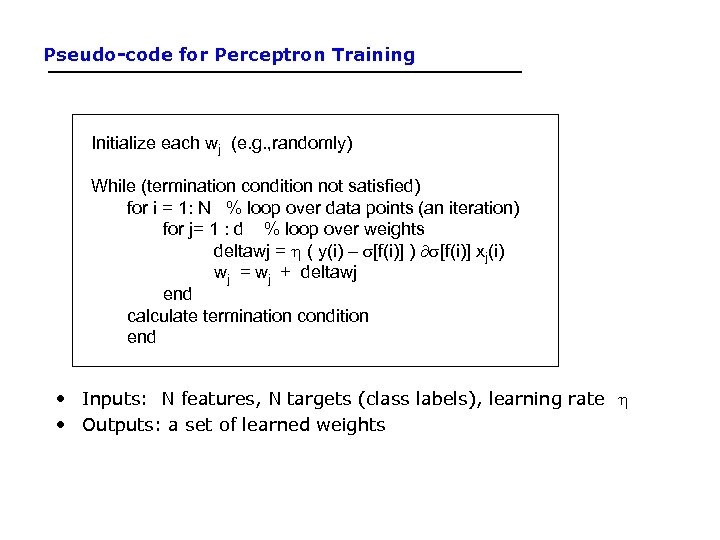Pseudo-code for Perceptron Training Initialize each wj (e. g. , randomly) While (termination condition not satisfied) for i = 1: N % loop over data points (an iteration) for j= 1 : d % loop over weights deltawj = h ( y(i) – s[f(i)] ) ¶s[f(i)] xj(i) wj = wj + deltawj end calculate termination condition end • Inputs: N features, N targets (class labels), learning rate h • Outputs: a set of learned weights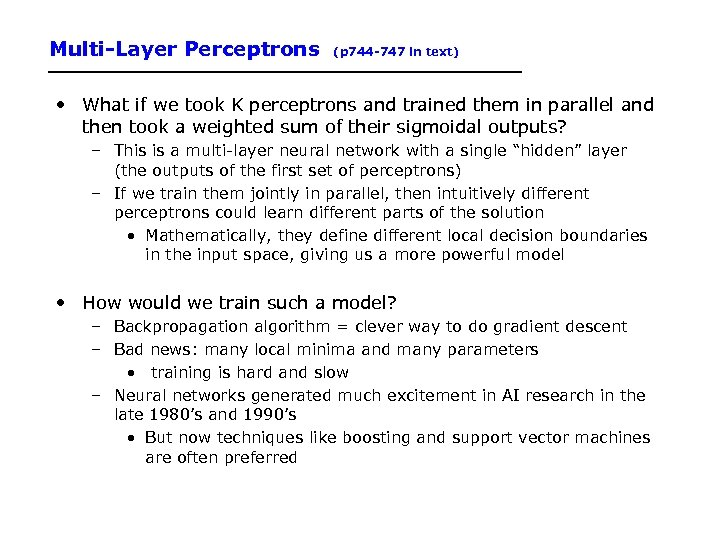Multi-Layer Perceptrons (p 744 -747 in text) • What if we took K perceptrons and trained them in parallel and then took a weighted sum of their sigmoidal outputs? – This is a multi-layer neural network with a single “hidden” layer (the outputs of the first set of perceptrons) – If we train them jointly in parallel, then intuitively different perceptrons could learn different parts of the solution • Mathematically, they define different local decision boundaries in the input space, giving us a more powerful model • How would we train such a model? – Backpropagation algorithm = clever way to do gradient descent – Bad news: many local minima and many parameters • training is hard and slow – Neural networks generated much excitement in AI research in the late 1980’s and 1990’s • But now techniques like boosting and support vector machines are often preferred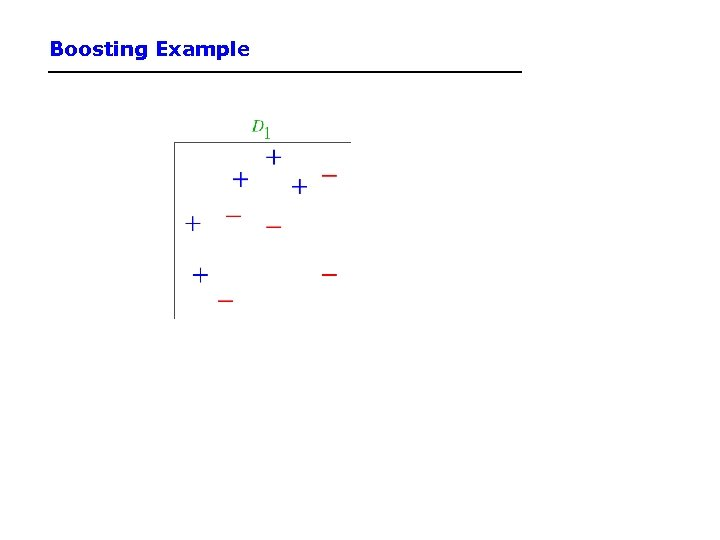Boosting Example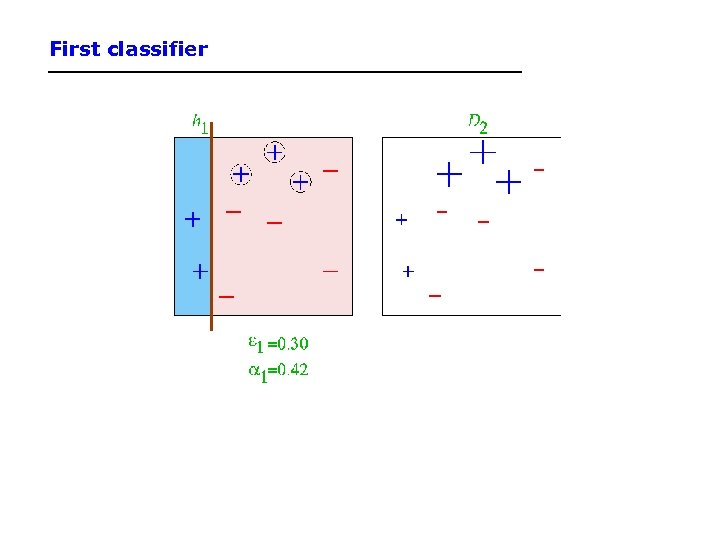First classifier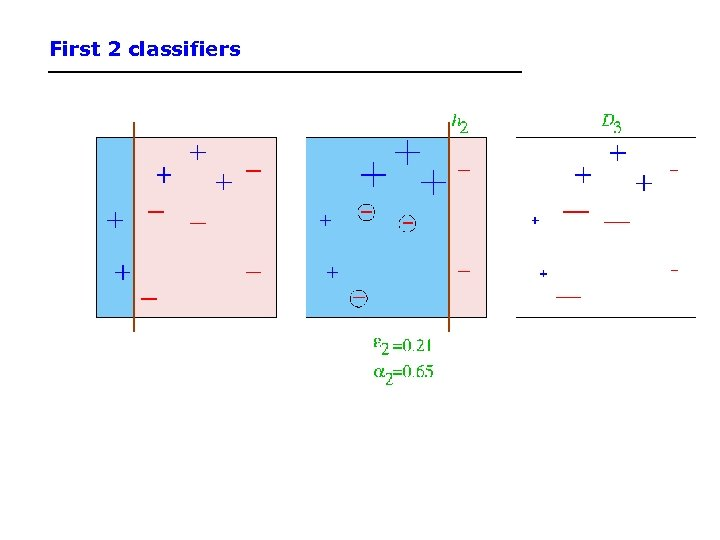First 2 classifiers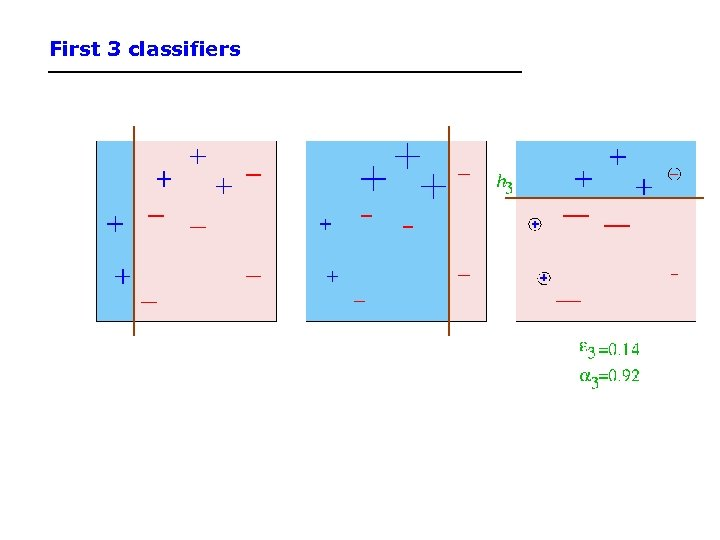First 3 classifiers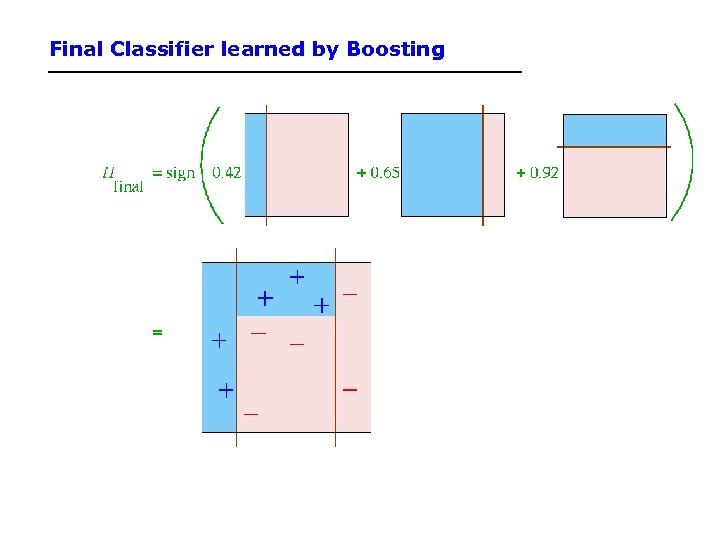Final Classifier learned by BoostingFinal Classifier learned by BoostingOutline • Dear Prof. Lathrop, Would you mind explain more about the statistic learning (chapter 20) while you are going through the material on today's class. It is very difficult for me to understand a few of my classmates have the same concern. Thank you – Reading assigned was Chapters 14. 1, 14. 2, plus: – 20. 1 -20. 3. 2 (3 rd ed. ) – 20. 1 -20. 7, but not the part of 20. 3 after “Learning Bayesian Networks” (2 nd ed. ) • Machine Learning • Probability and Uncertainty • Question & Answer – Iff time, Viola & Jones, 2004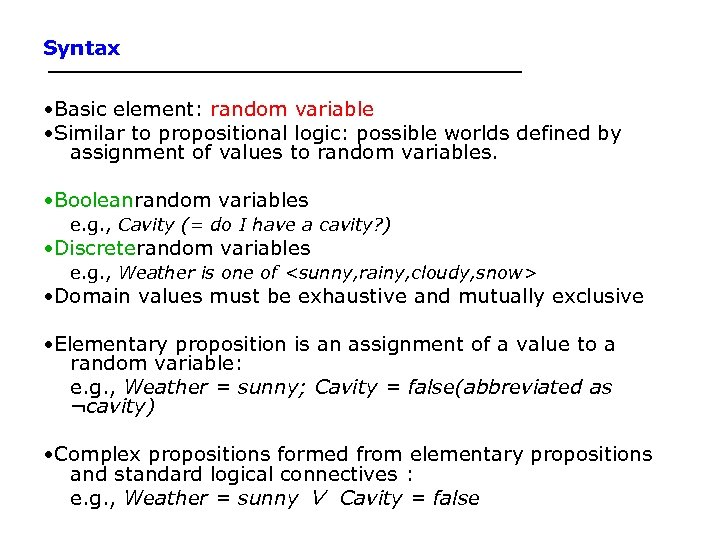Syntax • Basic element: random variable • Similar to propositional logic: possible worlds defined by assignment of values to random variables. • Booleanrandom variables e. g. , Cavity (= do I have a cavity? ) • Discreterandom variables e. g. , Weather is one of • Domain values must be exhaustive and mutually exclusive • Elementary proposition is an assignment of a value to a random variable: e. g. , Weather = sunny; Cavity = false(abbreviated as ¬cavity) • Complex propositions formed from elementary propositions and standard logical connectives : e. g. , Weather = sunny ∨ Cavity = false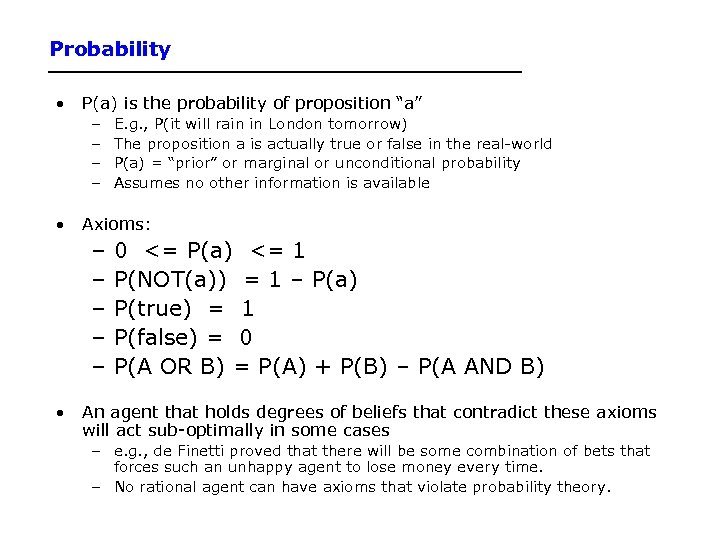Probability • P(a) is the probability of proposition “a” – – E. g. , P(it will rain in London tomorrow) The proposition a is actually true or false in the real-world P(a) = “prior” or marginal or unconditional probability Assumes no other information is available • Axioms: – – – 0 <= P(a) <= 1 P(NOT(a)) = 1 – P(a) P(true) = 1 P(false) = 0 P(A OR B) = P(A) + P(B) – P(A AND B) • An agent that holds degrees of beliefs that contradict these axioms will act sub-optimally in some cases – e. g. , de Finetti proved that there will be some combination of bets that forces such an unhappy agent to lose money every time. – No rational agent can have axioms that violate probability theory.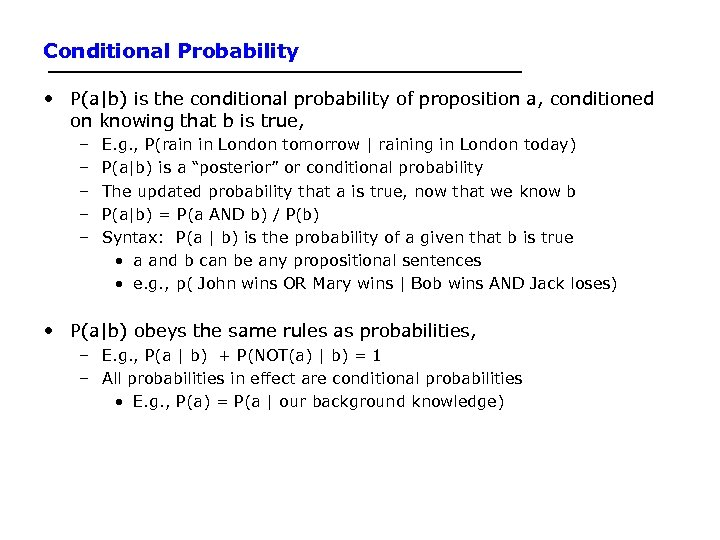Conditional Probability • P(a|b) is the conditional probability of proposition a, conditioned on knowing that b is true, – – – E. g. , P(rain in London tomorrow | raining in London today) P(a|b) is a “posterior” or conditional probability The updated probability that a is true, now that we know b P(a|b) = P(a AND b) / P(b) Syntax: P(a | b) is the probability of a given that b is true • a and b can be any propositional sentences • e. g. , p( John wins OR Mary wins | Bob wins AND Jack loses) • P(a|b) obeys the same rules as probabilities, – E. g. , P(a | b) + P(NOT(a) | b) = 1 – All probabilities in effect are conditional probabilities • E. g. , P(a) = P(a | our background knowledge)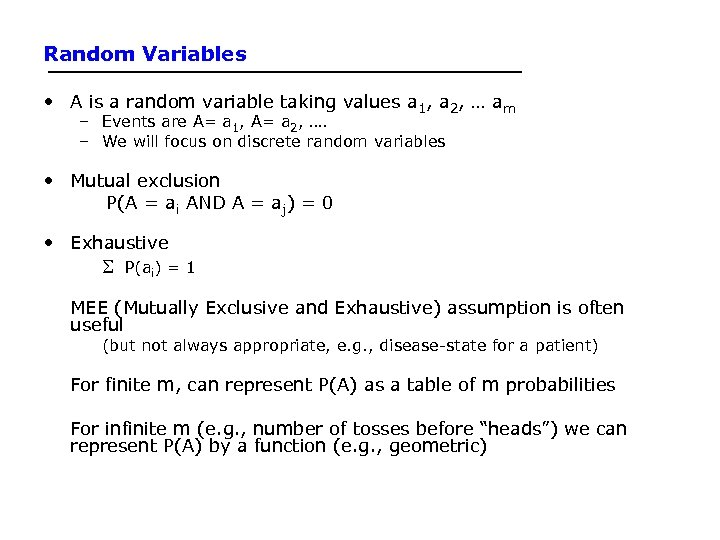Random Variables • A is a random variable taking values a 1, a 2, … am – Events are A= a 1, A= a 2, …. – We will focus on discrete random variables • Mutual exclusion P(A = ai AND A = aj) = 0 • Exhaustive P(ai) = 1 MEE (Mutually Exclusive and Exhaustive) assumption is often useful (but not always appropriate, e. g. , disease-state for a patient) For finite m, can represent P(A) as a table of m probabilities For infinite m (e. g. , number of tosses before “heads”) we can represent P(A) by a function (e. g. , geometric)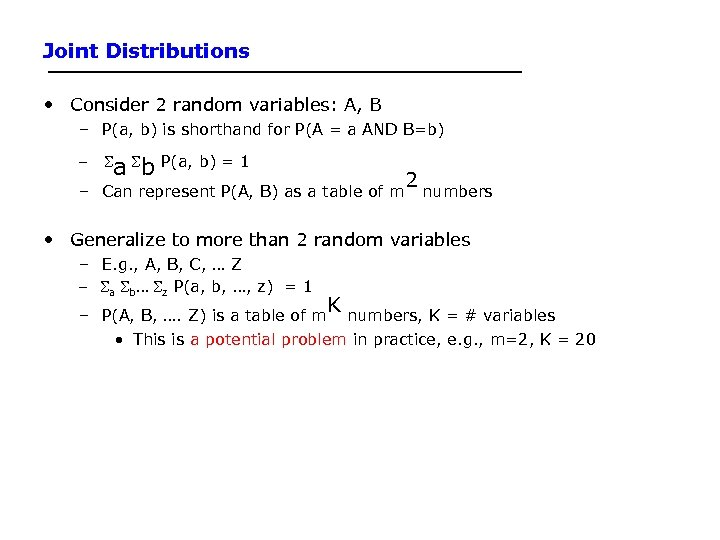Joint Distributions • Consider 2 random variables: A, B – P(a, b) is shorthand for P(A = a AND B=b) - a b P(a, b) = 1 – Can represent P(A, B) as a table of m 2 numbers • Generalize to more than 2 random variables – E. g. , A, B, C, … Z - a b… z P(a, b, …, z) = 1 K – P(A, B, …. Z) is a table of m numbers, K = # variables • This is a potential problem in practice, e. g. , m=2, K = 20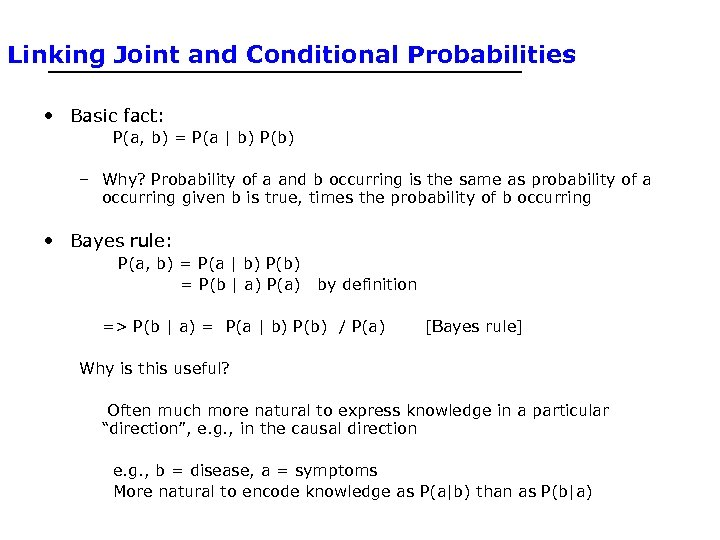Linking Joint and Conditional Probabilities • Basic fact: P(a, b) = P(a | b) P(b) – Why? Probability of a and b occurring is the same as probability of a occurring given b is true, times the probability of b occurring • Bayes rule: P(a, b) = P(a | b) P(b) = P(b | a) P(a) by definition => P(b | a) = P(a | b) P(b) / P(a) [Bayes rule] Why is this useful? Often much more natural to express knowledge in a particular “direction”, e. g. , in the causal direction e. g. , b = disease, a = symptoms More natural to encode knowledge as P(a|b) than as P(b|a)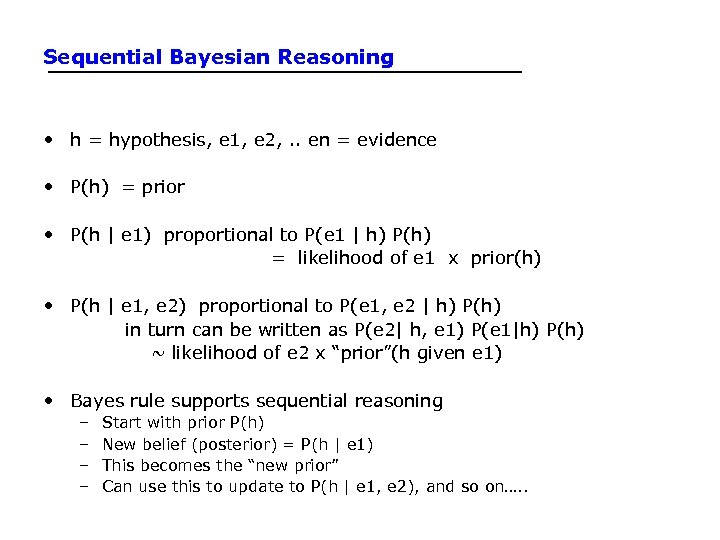Sequential Bayesian Reasoning • h = hypothesis, e 1, e 2, . . en = evidence • P(h) = prior • P(h | e 1) proportional to P(e 1 | h) P(h) = likelihood of e 1 x prior(h) • P(h | e 1, e 2) proportional to P(e 1, e 2 | h) P(h) in turn can be written as P(e 2| h, e 1) P(e 1|h) P(h) ~ likelihood of e 2 x “prior”(h given e 1) • Bayes rule supports sequential reasoning – – Start with prior P(h) New belief (posterior) = P(h | e 1) This becomes the “new prior” Can use this to update to P(h | e 1, e 2), and so on…. .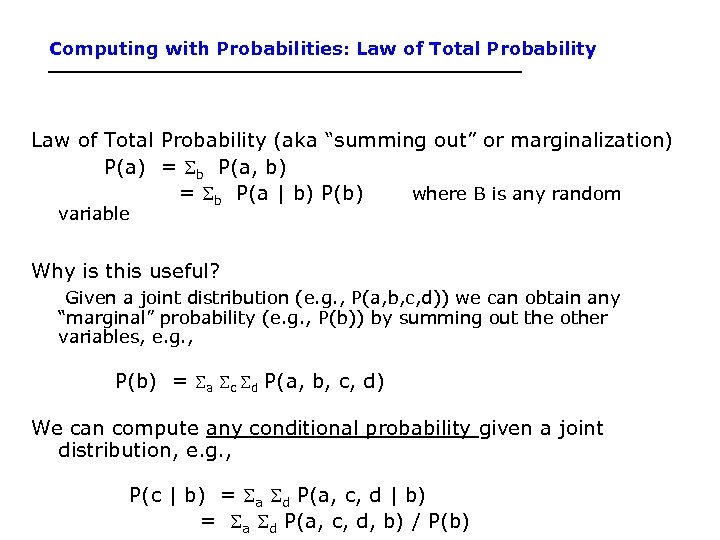Computing with Probabilities: Law of Total Probability (aka “summing out” or marginalization) P(a) = b P(a, b) = b P(a | b) P(b) where B is any random variable Why is this useful? Given a joint distribution (e. g. , P(a, b, c, d)) we can obtain any “marginal” probability (e. g. , P(b)) by summing out the other variables, e. g. , P(b) = a c d P(a, b, c, d) We can compute any conditional probability given a joint distribution, e. g. , P(c | b) = a d P(a, c, d, b) / P(b)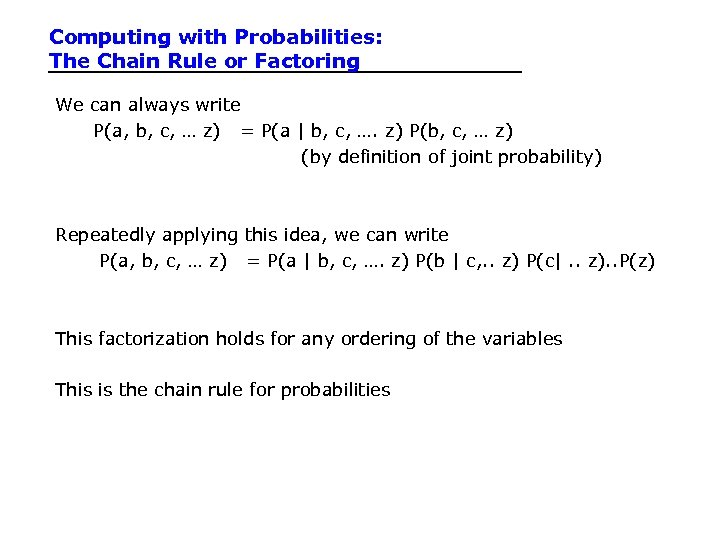Computing with Probabilities: The Chain Rule or Factoring We can always write P(a, b, c, … z) = P(a | b, c, …. z) P(b, c, … z) (by definition of joint probability) Repeatedly applying this idea, we can write P(a, b, c, … z) = P(a | b, c, …. z) P(b | c, . . z) P(c|. . z). . P(z) This factorization holds for any ordering of the variables This is the chain rule for probabilities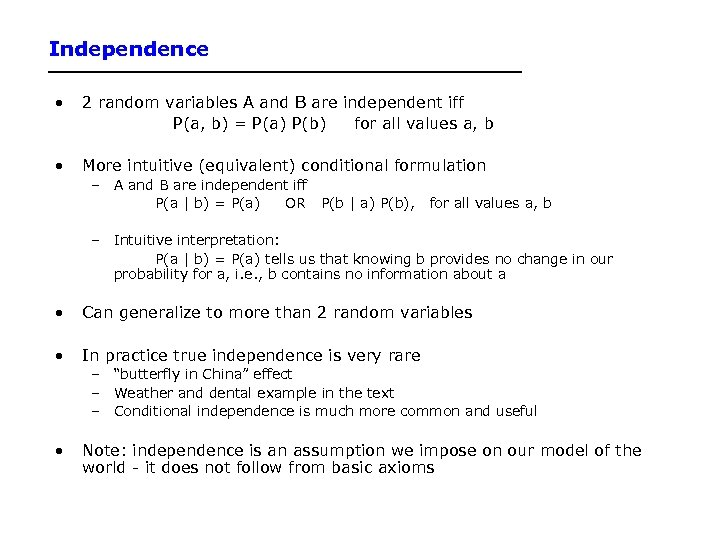Independence • 2 random variables A and B are independent iff P(a, b) = P(a) P(b) for all values a, b • More intuitive (equivalent) conditional formulation – A and B are independent iff P(a | b) = P(a) OR P(b | a) P(b), for all values a, b – Intuitive interpretation: P(a | b) = P(a) tells us that knowing b provides no change in our probability for a, i. e. , b contains no information about a • Can generalize to more than 2 random variables • In practice true independence is very rare – “butterfly in China” effect – Weather and dental example in the text – Conditional independence is much more common and useful • Note: independence is an assumption we impose on our model of the world - it does not follow from basic axioms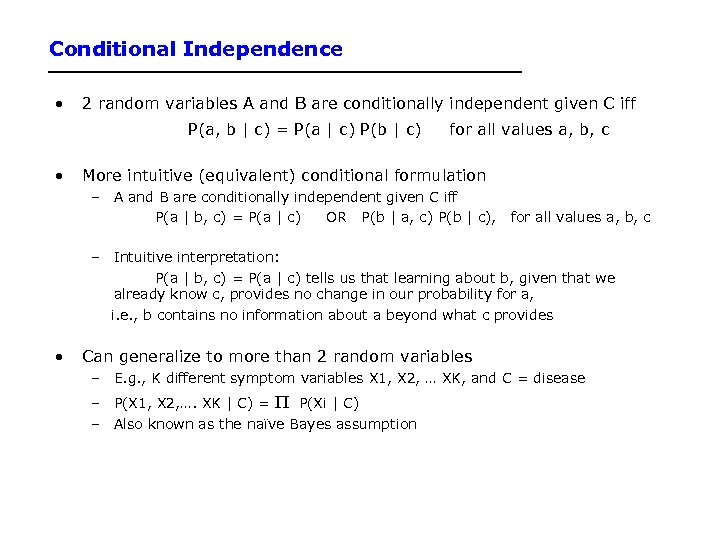Conditional Independence • 2 random variables A and B are conditionally independent given C iff P(a, b | c) = P(a | c) P(b | c) • for all values a, b, c More intuitive (equivalent) conditional formulation – A and B are conditionally independent given C iff P(a | b, c) = P(a | c) OR P(b | a, c) P(b | c), for all values a, b, c – Intuitive interpretation: P(a | b, c) = P(a | c) tells us that learning about b, given that we already know c, provides no change in our probability for a, i. e. , b contains no information about a beyond what c provides • Can generalize to more than 2 random variables – E. g. , K different symptom variables X 1, X 2, … XK, and C = disease – P(X 1, X 2, …. XK | C) = P(Xi | C) – Also known as the naïve Bayes assumptionOutline • Dear Prof. Lathrop, Would you mind explain more about the statistic learning (chapter 20) while you are going through the material on today's class. It is very difficult for me to understand a few of my classmates have the same concern. Thank you – Reading assigned was Chapters 14. 1, 14. 2, plus: – 20. 1 -20. 3. 2 (3 rd ed. ) – 20. 1 -20. 7, but not the part of 20. 3 after “Learning Bayesian Networks” (2 nd ed. ) • Machine Learning • Probability and Uncertainty • Question & Answer – Iff time, Viola & Jones, 2004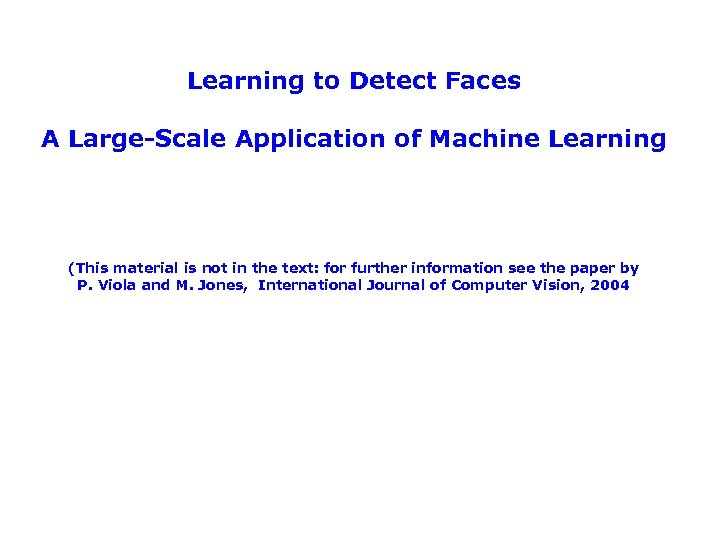Learning to Detect Faces A Large-Scale Application of Machine Learning (This material is not in the text: for further information see the paper by P. Viola and M. Jones, International Journal of Computer Vision, 2004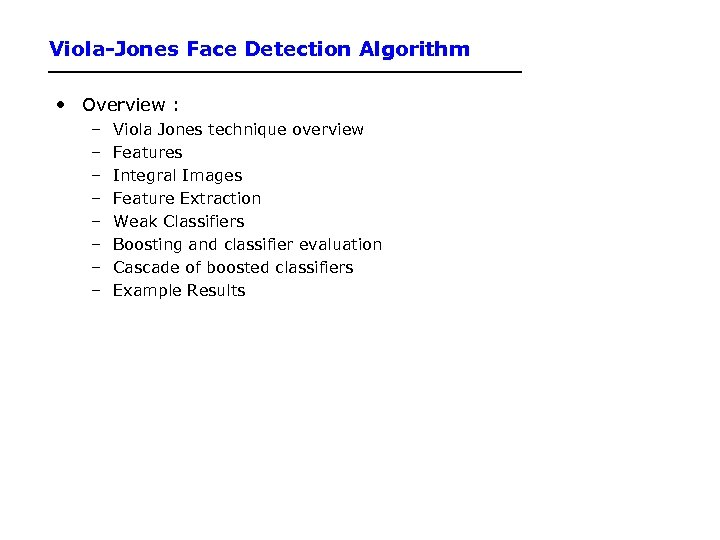Viola-Jones Face Detection Algorithm • Overview : – – – – Viola Jones technique overview Features Integral Images Feature Extraction Weak Classifiers Boosting and classifier evaluation Cascade of boosted classifiers Example Results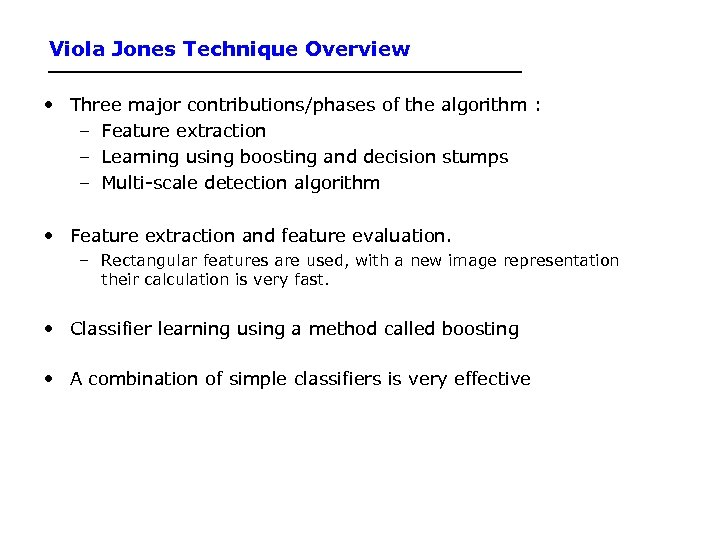Viola Jones Technique Overview • Three major contributions/phases of the algorithm : – Feature extraction – Learning using boosting and decision stumps – Multi-scale detection algorithm • Feature extraction and feature evaluation. – Rectangular features are used, with a new image representation their calculation is very fast. • Classifier learning using a method called boosting • A combination of simple classifiers is very effective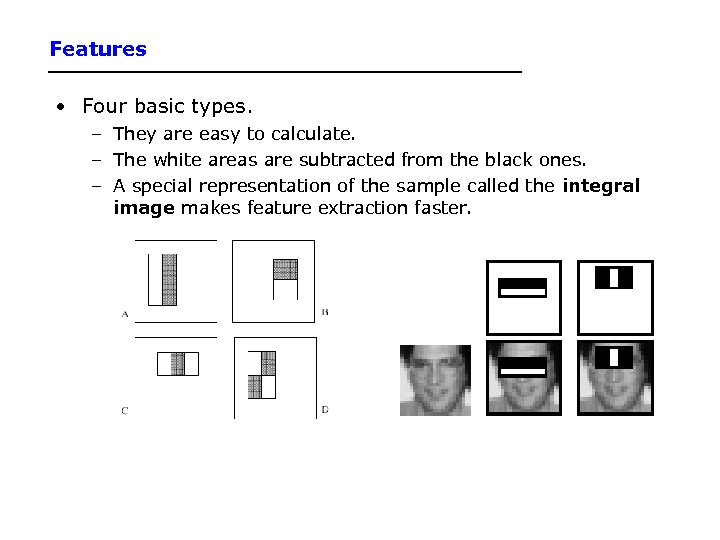Features • Four basic types. – They are easy to calculate. – The white areas are subtracted from the black ones. – A special representation of the sample called the integral image makes feature extraction faster.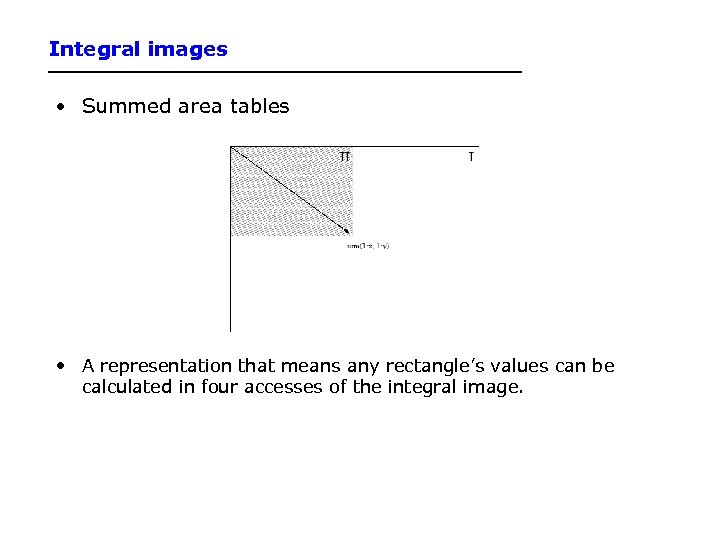Integral images • Summed area tables • A representation that means any rectangle’s values can be calculated in four accesses of the integral image.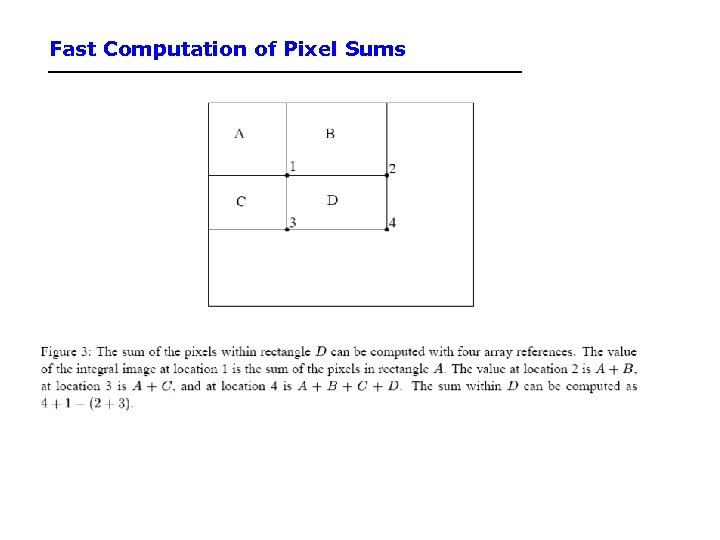Fast Computation of Pixel Sums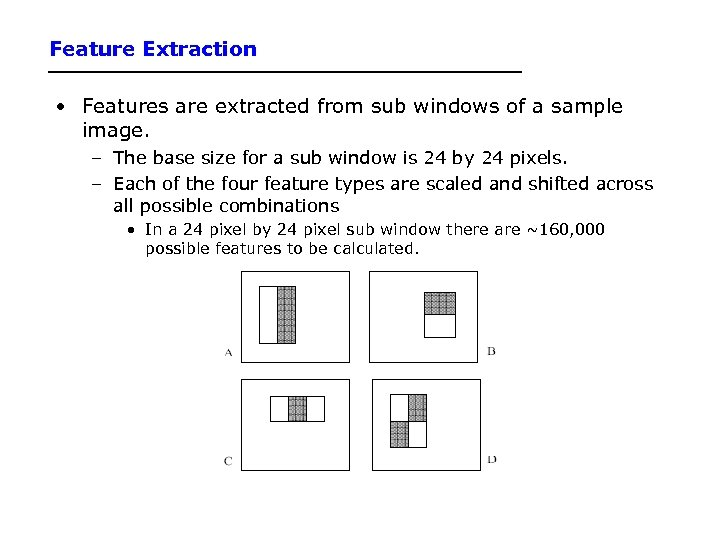Feature Extraction • Features are extracted from sub windows of a sample image. – The base size for a sub window is 24 by 24 pixels. – Each of the four feature types are scaled and shifted across all possible combinations • In a 24 pixel by 24 pixel sub window there are ~160, 000 possible features to be calculated.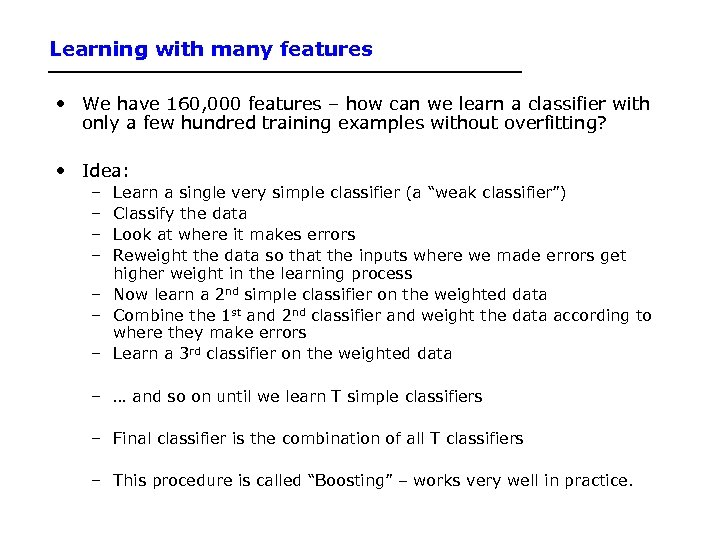Learning with many features • We have 160, 000 features – how can we learn a classifier with only a few hundred training examples without overfitting? • Idea: – – Learn a single very simple classifier (a “weak classifier”) Classify the data Look at where it makes errors Reweight the data so that the inputs where we made errors get higher weight in the learning process – Now learn a 2 nd simple classifier on the weighted data – Combine the 1 st and 2 nd classifier and weight the data according to where they make errors – Learn a 3 rd classifier on the weighted data – … and so on until we learn T simple classifiers – Final classifier is the combination of all T classifiers – This procedure is called “Boosting” – works very well in practice.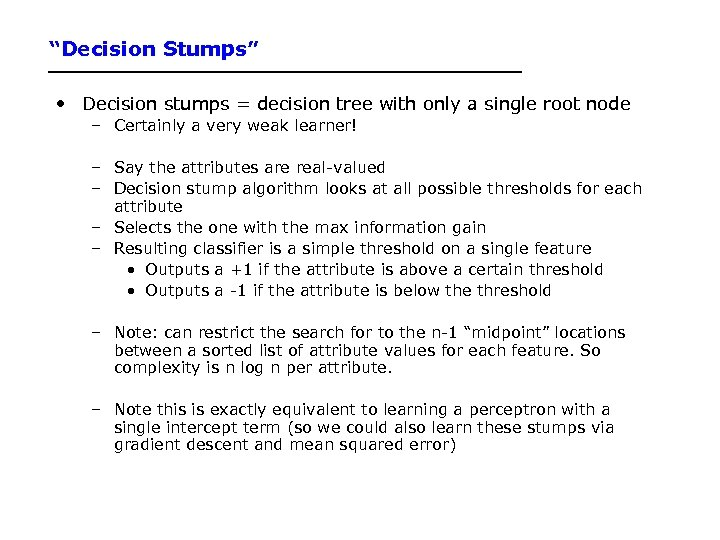“Decision Stumps” • Decision stumps = decision tree with only a single root node – Certainly a very weak learner! – Say the attributes are real-valued – Decision stump algorithm looks at all possible thresholds for each attribute – Selects the one with the max information gain – Resulting classifier is a simple threshold on a single feature • Outputs a +1 if the attribute is above a certain threshold • Outputs a -1 if the attribute is below the threshold – Note: can restrict the search for to the n-1 “midpoint” locations between a sorted list of attribute values for each feature. So complexity is n log n per attribute. – Note this is exactly equivalent to learning a perceptron with a single intercept term (so we could also learn these stumps via gradient descent and mean squared error)Boosting ExampleFirst classifierFirst 2 classifiersFirst 3 classifiersFinal Classifier learned by BoostingFinal Classifier learned by Boosting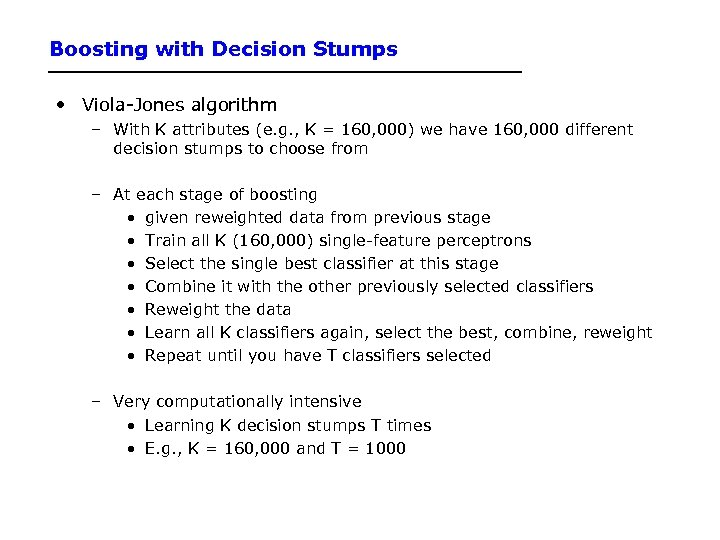Boosting with Decision Stumps • Viola-Jones algorithm – With K attributes (e. g. , K = 160, 000) we have 160, 000 different decision stumps to choose from – At each stage of boosting • given reweighted data from previous stage • Train all K (160, 000) single-feature perceptrons • Select the single best classifier at this stage • Combine it with the other previously selected classifiers • Reweight the data • Learn all K classifiers again, select the best, combine, reweight • Repeat until you have T classifiers selected – Very computationally intensive • Learning K decision stumps T times • E. g. , K = 160, 000 and T = 1000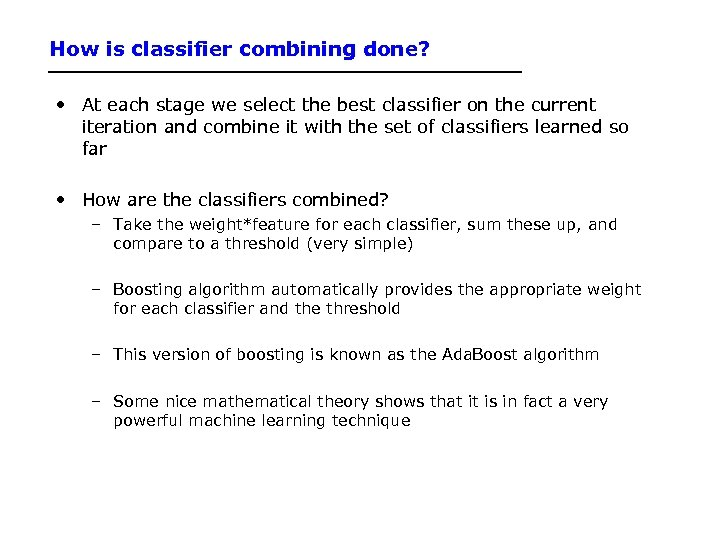How is classifier combining done? • At each stage we select the best classifier on the current iteration and combine it with the set of classifiers learned so far • How are the classifiers combined? – Take the weight*feature for each classifier, sum these up, and compare to a threshold (very simple) – Boosting algorithm automatically provides the appropriate weight for each classifier and the threshold – This version of boosting is known as the Ada. Boost algorithm – Some nice mathematical theory shows that it is in fact a very powerful machine learning technique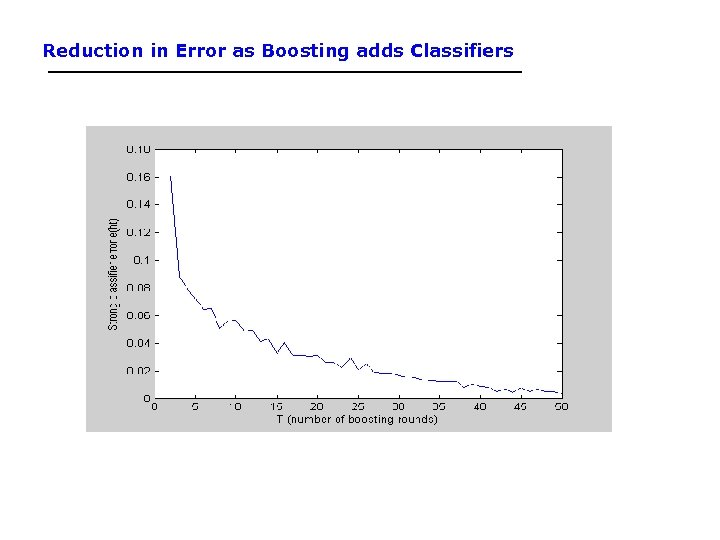Reduction in Error as Boosting adds Classifiers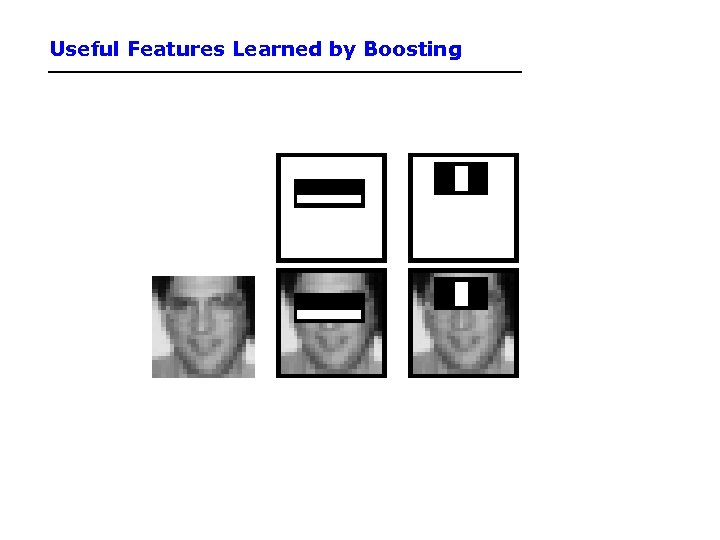Useful Features Learned by Boosting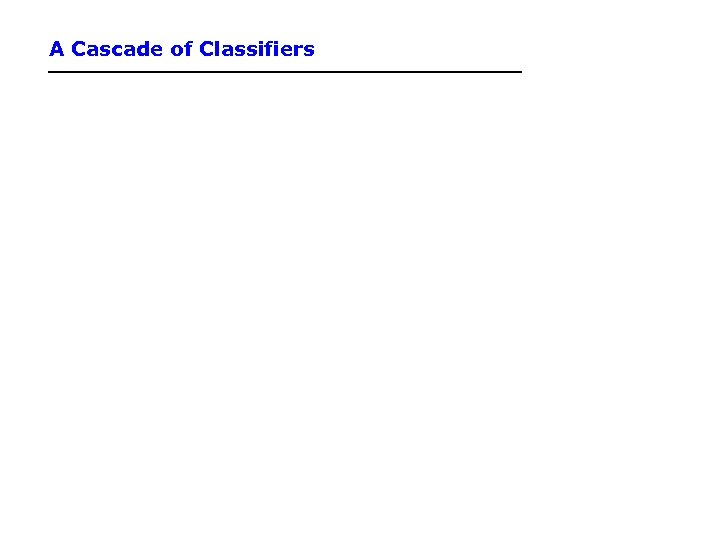A Cascade of Classifiers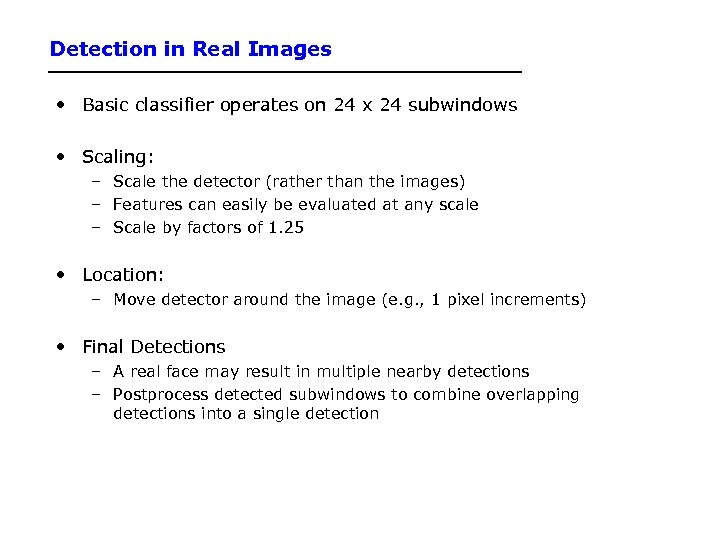Detection in Real Images • Basic classifier operates on 24 x 24 subwindows • Scaling: – Scale the detector (rather than the images) – Features can easily be evaluated at any scale – Scale by factors of 1. 25 • Location: – Move detector around the image (e. g. , 1 pixel increments) • Final Detections – A real face may result in multiple nearby detections – Postprocess detected subwindows to combine overlapping detections into a single detection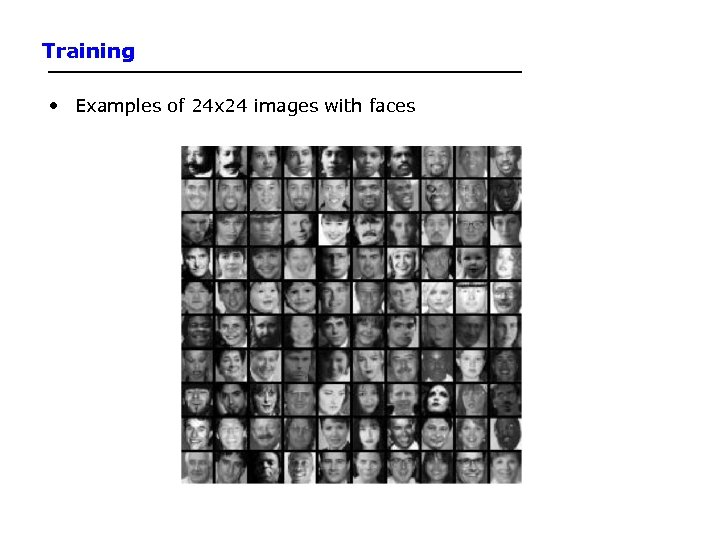Training • Examples of 24 x 24 images with faces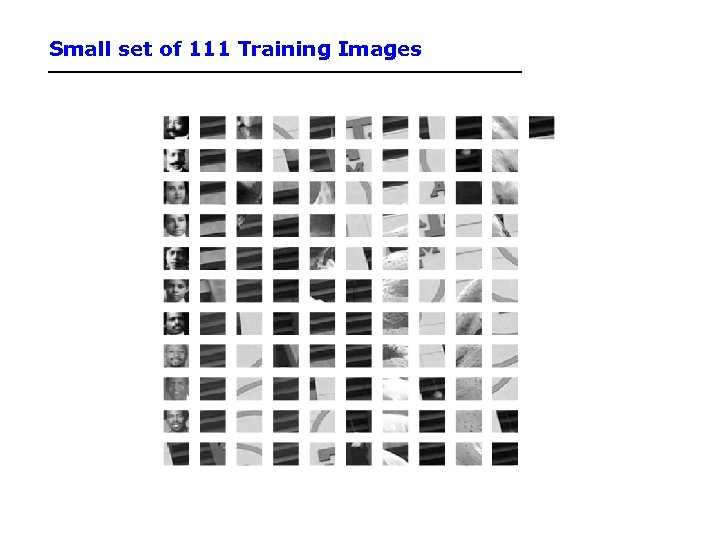Small set of 111 Training Images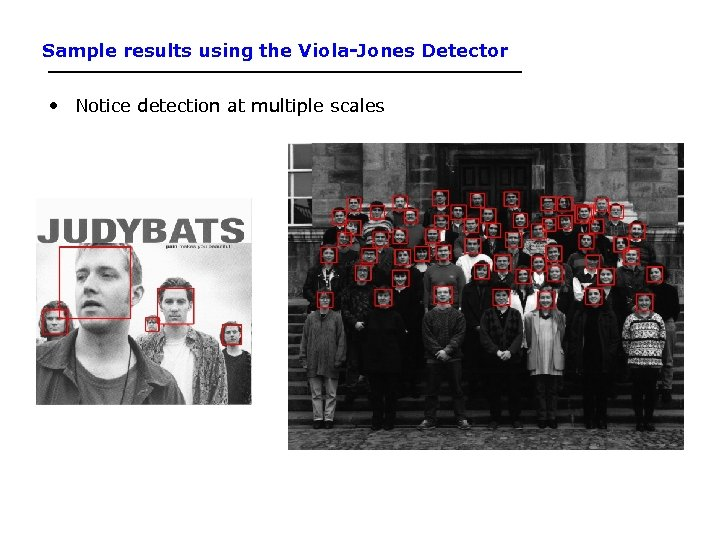Sample results using the Viola-Jones Detector • Notice detection at multiple scales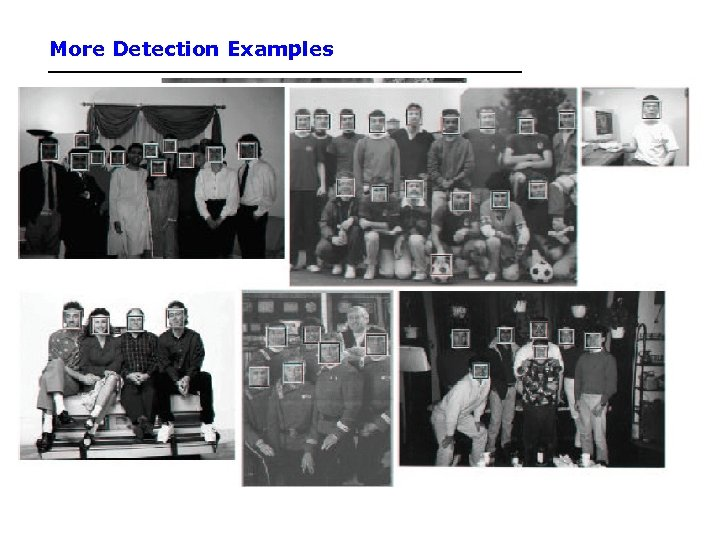More Detection Examples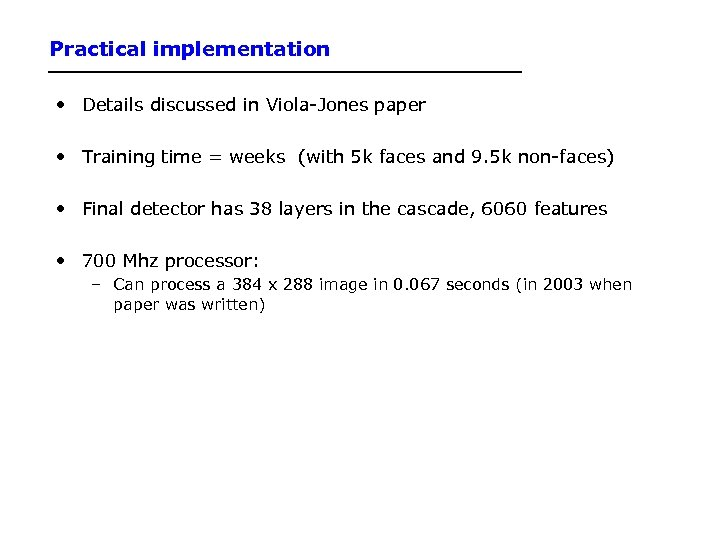Practical implementation • Details discussed in Viola-Jones paper • Training time = weeks (with 5 k faces and 9. 5 k non-faces) • Final detector has 38 layers in the cascade, 6060 features • 700 Mhz processor: – Can process a 384 x 288 image in 0. 067 seconds (in 2003 when paper was written)